# JP4774492B2 - Authentication system and remote distributed storage system - Google Patents

## Info

Publication number
JP4774492B2
JP4774492B2 JP2005514934A JP2005514934A JP4774492B2 JP 4774492 B2 JP4774492 B2 JP 4774492B2 JP 2005514934 A JP2005514934 A JP 2005514934A JP 2005514934 A JP2005514934 A JP 2005514934A JP 4774492 B2 JP4774492 B2 JP 4774492B2
Authority
JP
Japan
Prior art keywords
server
authentication
terminal device
data
value
Prior art date
Legal status (The legal status is an assumption and is not a legal conclusion. Google has not performed a legal analysis and makes no representation as to the accuracy of the status listed.)
Active
Application number
JP2005514934A
Other languages
Japanese (ja)
Other versions
JPWO2005041474A1 (en
Inventor

Original Assignee

Priority date (The priority date is an assumption and is not a legal conclusion. Google has not performed a legal analysis and makes no representation as to the accuracy of the date listed.)
Filing date
Publication date
Priority to JP2003367527 priority Critical
Priority to JP2003367527 priority
Application filed by 財団法人生産技術研究奨励会 filed Critical 財団法人生産技術研究奨励会
Priority to JP2005514934A priority patent/JP4774492B2/en
Priority to PCT/JP2004/015184 priority patent/WO2005041474A1/en
Publication of JPWO2005041474A1 publication Critical patent/JPWO2005041474A1/en
Application granted granted Critical
Publication of JP4774492B2 publication Critical patent/JP4774492B2/en
Application status is Active legal-status Critical
Anticipated expiration legal-status Critical

## Images

•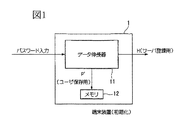•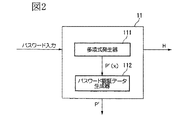•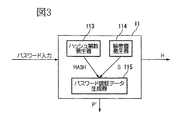•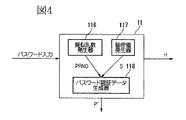•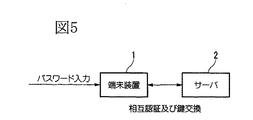•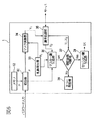•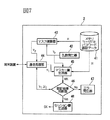••••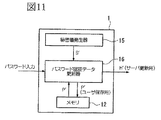•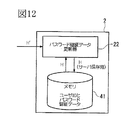•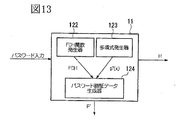•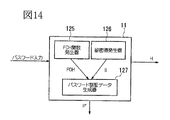•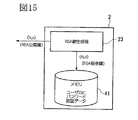•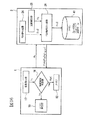•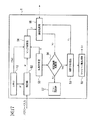•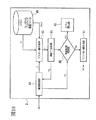•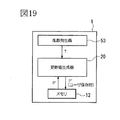•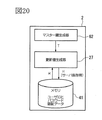•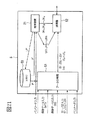•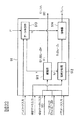•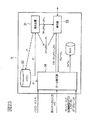•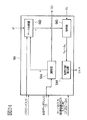•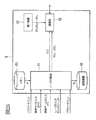•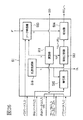••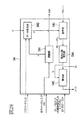## Classifications

• HELECTRICITY
• H04ELECTRIC COMMUNICATION TECHNIQUE
• H04LTRANSMISSION OF DIGITAL INFORMATION, e.g. TELEGRAPHIC COMMUNICATION
• H04L9/00Cryptographic mechanisms or cryptographic arrangements for secret or secure communication
• H04L9/08Key distribution or management, e.g. generation, sharing or updating, of cryptographic keys or passwords
• H04L9/0891Revocation or update of secret information, e.g. encryption key update or rekeying
• HELECTRICITY
• H04ELECTRIC COMMUNICATION TECHNIQUE
• H04LTRANSMISSION OF DIGITAL INFORMATION, e.g. TELEGRAPHIC COMMUNICATION
• H04L9/00Cryptographic mechanisms or cryptographic arrangements for secret or secure communication
• H04L9/30Public key, i.e. encryption algorithm being computationally infeasible to invert or user's encryption keys not requiring secrecy
• H04L9/3006Public key, i.e. encryption algorithm being computationally infeasible to invert or user's encryption keys not requiring secrecy underlying computational problems or public-key parameters
• H04L9/3026Public key, i.e. encryption algorithm being computationally infeasible to invert or user's encryption keys not requiring secrecy underlying computational problems or public-key parameters details relating to polynomials generation, e.g. generation of irreducible polynomials
• HELECTRICITY
• H04ELECTRIC COMMUNICATION TECHNIQUE
• H04LTRANSMISSION OF DIGITAL INFORMATION, e.g. TELEGRAPHIC COMMUNICATION
• H04L9/00Cryptographic mechanisms or cryptographic arrangements for secret or secure communication
• H04L9/32Cryptographic mechanisms or cryptographic arrangements for secret or secure communication including means for verifying the identity or authority of a user of the system or for message authentication, e.g. authorization, entity authentication, data integrity or data verification, non-repudiation, key authentication or verification of credentials
• H04L9/3226Cryptographic mechanisms or cryptographic arrangements for secret or secure communication including means for verifying the identity or authority of a user of the system or for message authentication, e.g. authorization, entity authentication, data integrity or data verification, non-repudiation, key authentication or verification of credentials using a predetermined code, e.g. password, passphrase or PIN
• HELECTRICITY
• H04ELECTRIC COMMUNICATION TECHNIQUE
• H04LTRANSMISSION OF DIGITAL INFORMATION, e.g. TELEGRAPHIC COMMUNICATION
• H04L9/00Cryptographic mechanisms or cryptographic arrangements for secret or secure communication
• H04L9/32Cryptographic mechanisms or cryptographic arrangements for secret or secure communication including means for verifying the identity or authority of a user of the system or for message authentication, e.g. authorization, entity authentication, data integrity or data verification, non-repudiation, key authentication or verification of credentials
• H04L9/3271Cryptographic mechanisms or cryptographic arrangements for secret or secure communication including means for verifying the identity or authority of a user of the system or for message authentication, e.g. authorization, entity authentication, data integrity or data verification, non-repudiation, key authentication or verification of credentials using challenge-response
• H04L9/3273Cryptographic mechanisms or cryptographic arrangements for secret or secure communication including means for verifying the identity or authority of a user of the system or for message authentication, e.g. authorization, entity authentication, data integrity or data verification, non-repudiation, key authentication or verification of credentials using challenge-response for mutual authentication
• HELECTRICITY
• H04ELECTRIC COMMUNICATION TECHNIQUE
• H04LTRANSMISSION OF DIGITAL INFORMATION, e.g. TELEGRAPHIC COMMUNICATION
• H04L2209/00Additional information or applications relating to cryptographic mechanisms or cryptographic arrangements for secret or secure communication H04L9/00

## Description

The present invention relates to an authentication system that is strong against leakage of information related to authentication, and a remote distributed storage system that can safely store data using this authentication system.
This application claims priority with respect to Japanese Patent Application No. 2003-367527 for which it applied on October 28, 2003, and uses the content here.

Conventionally, as a method of performing authentication between the user's terminal device and the server, if the user ID and the password known only by the user are input from the terminal device and match the information stored on the server side, the authentication is valid. There is known a method for authenticating that the user is a valid user.
However, in this method, if such information is stolen illegally in the communication path between the terminal device and the server, unauthorized use is easily permitted, so SSL (Reference 1), TLS (Reference 2) In general, information is transmitted and received using an encryption technique such as SSH (Document 3). In this method, authentication is performed using a password, a secret value, and a public value.

(Reference 1) A.1. Frier, P.M. Karlton, and P.M. Kocher. The SSL 3.0 Protocol. Netscape Communications Corp. , 1996, http: // wp. netscape. com / eng / ss13 /
(Reference 2) IETF (Internet Engineering Task Force). Transport Layer security (tls) Charter. http: // www. ietf. org / html. charters / tls-charter. html
(Reference 3) IETF (Internet Engineering Task Force). Secure Shell (secsh) Charter. http: // www. ietf. org / html. charters / secsh-charter. html

However, the methods shown in Non-Patent Documents 1 to 3 can obtain a password by offline analysis work when information encrypted with a password from the terminal device side of the user or password authentication data leaks from the server side. There is a problem that it ends up. The method of repeatedly entering the password online to the server can take measures such as denying access according to the number of wrong passwords, but it can take preventive measures for offline analysis work. There is also a problem that it cannot be done. In addition, when a password is leaked, there is a problem that data stored in a system that can log in with this password is also leaked.

An object of the present invention is to provide an authentication system that is strong against leakage of information and can safely exchange encryption keys.
An object of the present invention is to provide a remote distributed storage system that can securely store data using an authentication system according to the present invention.

The gist of the present invention is an authentication program that operates on a terminal device in an authentication system that performs mutual authentication between a terminal device and a server, and a password for server registration based on a password determined in advance by a user Data decompression process for obtaining authentication data H and authentication information P ′ for user storage, storage process for storing authentication information P ′ obtained by the data decompression process in advance, and authentication stored by the storage process A combination process for obtaining a value P by a predetermined calculation formula using the information P ′ and a password input at the time of authentication as input, and a value Y1 by a predetermined calculation formula for inputting the value P and a random number generated therein, A mask calculation process to be transmitted to the server, the value P, an internally generated random number, and a value Y2 received from the server as inputs. A master key generation process for obtaining a value MK using an equation, a value V1 obtained by a predetermined calculation formula using the value MK as an input, and transmitted to a server, and a value V2 received from the server and the value MK as an input The computer compares and verifies with the value V2 based on the calculation formula, and makes the computer perform an authentication result determination process for authenticating the server if they match.

The gist of the present invention is an authentication program that operates on a server in an authentication system that performs mutual authentication between a terminal device and a server, and stores the password authentication data H in advance and the storage process The password authentication data H and the random number generated inside are input, a value Y2 is obtained by a predetermined calculation formula, transmitted to the terminal device, and the password authentication data H and the password authentication data H are generated inside. A master key generation process for obtaining a value MK by a predetermined calculation formula using a random number and the value Y1 received from the terminal device as an input, and a value V2 for a predetermined calculation formula by using the value MK as an input, and transmitting to the terminal device The value V1 received from the terminal device and the value MK are input and compared with the value V1 according to a predetermined calculation formula. And an authentication result of the determination process of authenticating the terminal device causing a computer when.

The gist of the present invention is an authentication program that operates on a terminal device in an authentication system that performs mutual authentication between a terminal device and a server, and a password for server registration based on a password determined in advance by a user Data decompression processing for obtaining authentication data H and user storage authentication information P ′, authentication information P ′ obtained by the data decompression processing, and RSA public key (N, e) obtained by RSA key generation processing are stored in advance. Storage processing, authentication processing P ′ stored by the storage processing, and a combination processing for obtaining a value W by a predetermined calculation formula using the password input at the time of authentication as input, and the value W and the storage processing Using the stored RSA public key (N, e) and the internally generated random number T as an input, a value Z is obtained by a predetermined calculation formula and sent to the server. A mask calculation process, an authentication result determination process for authenticating the server when the value V2 received from the server and the value V2 according to a predetermined calculation formula are compared and matched, and the random number T is A value V1 is obtained by a predetermined calculation formula as an input, and a verifier generation process to be transmitted to the server is performed by the computer.

The gist of the present invention is an authentication program that operates on a server in an authentication system that performs mutual authentication between a terminal device and a server, and obtains an RSA public key (N, e) and an RSA private key (N, d). RSA key generation processing, RSA private key (N, d) obtained by the RSA key generation processing and storage processing for storing password authentication data H in advance, RSA private key stored by the storage processing ( N, d), the password authentication data H and the value Z received from the terminal device as input, a master key generation process for obtaining a value T by a predetermined calculation formula, and the value T as an input to obtain a value V2 by the predetermined calculation formula The verifier generation process to be obtained and transmitted to the terminal device, the value V1 received from the terminal device and the value T as input and compared with the value V1 by a predetermined calculation formula, and matched To perform the authentication result determination process of authenticating the terminal device if the computer.

The gist of the present invention operates on a terminal device in a remote distributed storage system that performs mutual authentication between a terminal device and a plurality of servers and distributes and stores data to be stored in the terminal device in the server. A remote distributed storage program comprising: a data expansion process for obtaining password authentication data H for server registration and authentication information P ′ for user storage based on a password predetermined by a user; and the data expansion process A storage process for preliminarily storing the obtained authentication information P ′, a combination process for obtaining the value P by a predetermined calculation formula using the authentication information P ′ read from the storage process and the password input at the time of authentication as inputs, A mask calculation process in which a value Y1 is obtained by a predetermined calculation formula using the value P and a random number generated therein as an input, and transmitted to the server; Master key generation processing for obtaining a value MK by a predetermined calculation formula using P and a random number generated internally and the value Y2 received from the server as input, and obtaining a value V1 by a predetermined calculation formula using the value MK as an input, A value V2 and a value V1 received from the server are compared and collated, and the authentication result determination process for authenticating the server when they match, and when the server is authenticated, the session key SK is transmitted to the server. Session key generation processing for generating the same number of data, data division processing for dividing the storage target data to obtain the same number of divided data as the number of authenticated servers, and identifying each of the divided data and the storage target data Data storage processing for encrypting the identification information to be transmitted using the session key SK shared with the storage destination server and transmitting the encrypted information to each server; It receives the divided data from each server to split data is stored, the remote distributed storage program characterized by causing a data restoration process for restoring the data of the storage target in a computer.

The gist of the present invention is that a remote operation that operates on a server in a remote distributed storage system that performs mutual authentication between a terminal device and a plurality of servers and distributes and stores data to be stored in the terminal device in the server. A distributed storage program for storing password authentication data H obtained by data decompression processing in advance, password authentication data H read from the storage processing, and a random number generated therein as input. A value Y2 is obtained by an equation, and a mask calculation process to be transmitted to the terminal device, the password authentication data H, a random number generated inside, and a value Y1 received from the terminal device are input, and a value MK is obtained by a predetermined equation. A master key generation process to be obtained and a value V2 is obtained by a predetermined calculation formula using the value MK as an input and sent to the terminal device At the same time, the value V1 and the value V2 received from the terminal device are compared and collated, and the authentication result determination process for authenticating the terminal device when they match, and the session key is generated when the terminal device is authenticated. Session key generation processing, data reception processing for receiving the divided data received from the terminal device, data storage processing for storing the divided data, and reading the divided data stored in the data storage processing and transmitting it to the terminal device A remote distributed storage program that causes a computer to perform data transmission processing.

<First embodiment>
Hereinafter, preferred embodiments of the present invention will be described with reference to the drawings. However, the present invention is not limited to the following embodiments, and for example, the constituent elements of these embodiments may be appropriately combined.
This authentication system is a system for securing the same session key while mutually authenticating each other between a user terminal device and a server authentication device.
Here, symbols used in the following description will be described.
p and q are prime numbers and have a relationship of q | p−1. q | p-1 means that q is a value that can divide p-1. Further, g and h are generators of a finite field (group) of order q on modp G = {g j mod p: 0 ≦ j <q} (can be configured in the same manner on a group on an elliptic curve) . Here, “g j mod p” means a remainder (Reminder) obtained by dividing a value obtained by raising g to the power of j by p. Here, g is (1 <g <p−1, g q = 1 modp, g j ≠ 1 mod p (0 <j <q)), and h is h = g a modp. That is, p and q indicate a calculation system (element characteristic). For example, H = h x modp (0 <x <q) and x is secret information (that is, when H is given, it is mathematically difficult to find x = log h H; Discrete logarithm problem for h). The random number generated from the random number generator randomly generates Rε (Z / qZ) * . Here, (Z / qZ) * indicates a set of {1, 2,..., Q}. N indicates the length of the password. Also, ‖ means that the values are concatenated.

<Initialization of terminal device>
When the user wants to register personally with the server, the user initializes his terminal device. FIG. 1 is a block diagram showing a configuration of initialization processing of a user terminal device. In the initialization, when the user inputs a password, the data decompressor 11 generates password authentication data H for server registration and a value P ′ for user storage, and the password authentication data H is transferred to the server. The value P ′ is stored in the memory 12. Here, the data decompressor 11 can be composed of a polynomial, a polynomial and a hash function, a hash function, a pseudo random number generator, or the like.

(1) When using a polynomial (1)
First, a case (part 1) in which a polynomial is used will be described with reference to FIG.
First, a polynomial generator 111 randomly generates a polynomial. At this time, if the number of servers to be registered is one, a first order polynomial (p ′ (x) = α 1 · xmodq) having x as a variable is used, and if the number of servers is n, an nth order polynomial (p ′ ( x) = α 1 · x + α 2 · x 2 +... + α n · x n modq). Here, α is randomly selected from (Z / qZ) * .
For example, in the case of one server, p ′ (x) is p ′ (x) = α 1 · xmodq. Here, the user inputs the password he / she remembers (for example, “Pooh93”). When the polynomial and the user password are input, the password authentication data generator 112 generates password authentication data H. The password authentication data H can be calculated by, for example, H = h p ′ (1) + Pooh93 modp. Here, p ′ (1) is a value calculated by inserting a server ID (for example, “1”) in place of x in p ′ (x). The password authentication data H needs to be notified safely by the user by handing it directly to the server, sending it by mail, or notifying it by telephone. A memory 12 inside the user's terminal device stores and saves the polynomial P ′ = p ′ (x) generated from the polynomial generator.

(2) When using a polynomial (part 2)
Next, with reference to FIG. 2, the case where a polynomial is used (part 2) will be described.
First, a polynomial generator 111 randomly generates a polynomial. At this time, if the number of servers to be registered is one, a first order polynomial (p ′ (x) = α 1 · xmodq) having x as a variable is used, and if the number of servers is n, an nth order polynomial (p ′ ( x) = α 1 · x + α 2 · x 2 +... + α n · x n modq). Here, α is randomly selected from (Z / qZ) * .
For example, in the case of one server, p ′ (x) is p ′ (x) = α 1 · xmodq. Here, the user inputs the password he / she remembers (for example, “Pooh93”). When the polynomial and the user password are input, the password authentication data generator 112 generates password authentication data H. The password authentication data H can be calculated by, for example, H = p (1) = p ′ (1) + Pooh93modq. Here, p ′ (1) is a value calculated by inserting a server ID (for example, “1”) in place of x in p ′ (x). The password authentication data H needs to be notified safely by the user by handing it directly to the server, sending it by mail, or notifying it by telephone. A memory 12 inside the user's terminal device stores and saves the polynomial P ′ = p ′ (x) generated from the polynomial generator.

(3) When using polynomial and hash function (1)
Next, with reference to FIG. 8, a case (part 1) in which a polynomial and a hash function are used will be described.
First, a polynomial generator 119 randomly generates a polynomial. At this time, if the number of servers to be registered is one, a first order polynomial (p ′ (x) = α 1 · xmodN) having x as a variable is used, and if the number of servers is n, an nth order polynomial (p ′ ( x) = α 1 · x + α 2 · x 2 +... + α n · x n mod N ). Here, α is randomly selected from (Z / qZ) * .
For example, in the case of one server, p ′ (x) is p ′ (x) = α 1 · xmodN. The hash function generator 120 generates a hash function HASH at random. HASH is a one-way hash function. Here, the user inputs the password he / she remembers (for example, “Pooh93”). When the polynomial, the hash function, and the user password are input, the password authentication data generator 121 generates password authentication data H. The password authentication data H can be calculated by, for example, H = h p (1) modp. Here, p (1) is calculated by p (1) = p ′ (1) + HASH (Pooh93‖ID (U) ‖ID (S)) modN. Here, ID (U) and ID (S) represent the IDs of the user and the server, respectively. Here, p ′ (1) is a value calculated by inserting “1” in place of x in p ′ (x).
For example, when the number of servers to be registered is n, the password authentication data generator 121 generates password authentication data H for the i-th server. The password authentication data H can be calculated by, for example, H = h p (i) modp. Here, p (i) is calculated by p (i) = p ′ (i) + HASH (Pooh93‖ID (U) ‖ID (S)) modN. Here, ID (U) and ID (S) represent the IDs of the user and the i-th server, respectively. Here, p ′ (i) is a value calculated by inserting “i” instead of x in the n-th order polynomial p ′ (x).
The password authentication data H needs to be notified safely by the user by handing it directly to the server, sending it by mail, or notifying it by telephone. The memory 12 inside the user terminal device combines the polynomial p ′ (x) generated from the polynomial generator and the hash function HASH generated from the hash function generator together with P ′ = (p ′ (x), Store and save as HASH).

(4) When using polynomial and hash function (2)
Next, with reference to FIG. 8, a case (part 2) in which a polynomial and a hash function are used will be described.
First, a polynomial generator 119 randomly generates a polynomial. At this time, if the number of servers to be registered is one, a first order polynomial (p ′ (x) = α 1 · xmodN) having x as a variable is used, and if the number of servers is n, an nth order polynomial (p ′ ( x) = α 1 · x + α 2 · x 2 +... + α n · x n mod N ). Here, α is randomly selected from (Z / qZ) * .
For example, in the case of one server, p ′ (x) is p ′ (x) = α 1 · xmodN. The hash function generator 120 generates a hash function HASH at random. HASH is a one-way hash function. Here, the user inputs the password he / she remembers (for example, “Pooh93”). When the polynomial, the hash function, and the user password are input, the password authentication data generator 121 generates password authentication data H. The password authentication data H can be calculated by, for example, H = p (1) = p ′ (1) + HASH (Pooh93‖ID (U) ‖ID (S)) modN. Here, ID (U) and ID (S) represent the IDs of the user and the server, respectively. Here, p ′ (1) is a value calculated by inserting “1” in place of x in p ′ (x).
For example, when the number of servers to be registered is n, the password authentication data generator 121 generates password authentication data H for the i-th server. The password authentication data H can be calculated by, for example, H = p (i) = p ′ (i) + HASH (Pooh93‖ID (U) ‖ID (S)) modN. Here, ID (U) and ID (S) represent the IDs of the user and the i-th server, respectively. Here, p ′ (i) is a value calculated by inserting “i” instead of x in the n-th order polynomial p ′ (x).
The password authentication data H needs to be notified safely by the user by handing it directly to the server, sending it by mail, or notifying it by telephone. The memory 12 inside the user terminal device combines the polynomial p ′ (x) generated from the polynomial generator and the hash function HASH generated from the hash function generator together with P ′ = (p ′ (x), Store and save as HASH).

(5) When using a hash function (part 1)
Next, with reference to FIG. 3, the case where the hash function is used (part 1) will be described.
First, a hash function HASH is randomly generated by the hash function generator 113. The secret value generator 114 also randomly generates a secret value S. Here, the user inputs the password he / she remembers (for example, “Pooh93”). When the hash function HASH, the secret value S, and the user password are input, the password authentication data generator 115 generates password authentication data H. The password authentication data H can be calculated by, for example, H = h HASH (S‖Pooh93‖ID (U) ‖ID (S)) modp. Here, ID (U) and ID (S) represent the IDs of the user and the server, respectively. The password authentication data H needs to be notified safely by the user by handing it directly to the server, sending it by mail, or notifying it by telephone. The memory 12 inside the user terminal device stores and stores the hash function HASH generated from the hash function generator 113 and the secret value generator 114 and the secret value S together as P ′ = (S, HASH). To do.

(6) When using a hash function (part 2)
Next, with reference to FIG. 3, the case where the hash function is used (part 2) will be described.
First, a hash function HASH is randomly generated by the hash function generator 113. The secret value generator 114 also randomly generates a secret value S. Here, the user inputs the password he / she remembers (for example, “Pooh93”). When the hash function HASH, the secret value S, and the user password are input, the password authentication data generator 115 generates password authentication data H. The password authentication data H can be calculated by, for example, H = HASH (S‖Pooh93‖ID (U) ‖ID (S)) modq. Here, ID (U) and ID (S) represent the IDs of the user and the server, respectively. The password authentication data H needs to be notified safely by the user by handing it directly to the server, sending it by mail, or notifying it by telephone. The memory 12 inside the user terminal device stores and stores the hash function HASH generated from the hash function generator 113 and the secret value generator 114 and the secret value S together as P ′ = (S, HASH). To do.

(7) When using a pseudo-random number generator (1)
Next, with reference to FIG. 4, the case (the 1) using a pseudorandom number generator is demonstrated. First, the pseudorandom number generator 116 randomly generates a pseudorandom function PRNG. The secret value generator 117 also randomly generates a secret value S. Here, the user inputs the password he / she remembers (for example, “Pooh93”). In response to the input of the pseudorandom function PRNG, the secret value S, and the user password, the password authentication data generator 118 generates password authentication data H. The password authentication data H can be calculated by, for example, H = h PRNG (S‖Pooh93‖ID (U) ‖ID (S)) modp. Here, ID (U) and ID (S) represent the IDs of the user and the server, respectively. The password authentication data H needs to be notified safely by the user by handing it directly to the server, sending it by mail, or notifying it by telephone. The memory 12 inside the user terminal device stores the pseudo random number function PRNG generated from the pseudo random number generator 116 and the secret value generator 117 and the secret value S together as P ′ = (S, PRNG). save.

(8) When using a pseudo random number generator (2)
Next, with reference to FIG. 4, the case where the pseudo random number generator is used (part 2) will be described. First, the pseudorandom number generator 116 randomly generates a pseudorandom function PRNG. The secret value generator 117 also randomly generates a secret value S. Here, the user inputs the password he / she remembers (for example, “Pooh93”). In response to the input of the pseudorandom function PRNG, the secret value S, and the user password, the password authentication data generator 118 generates password authentication data H. The password authentication data H can be calculated by, for example, H = PRNG (S‖Pooh93‖ID (U) ‖ID (S)) modq. Here, ID (U) and ID (S) represent the IDs of the user and the server, respectively. The password authentication data H needs to be notified safely by the user by handing it directly to the server, sending it by mail, or notifying it by telephone. The memory 12 inside the user terminal device stores the pseudo random number function PRNG generated from the pseudo random number generator 116 and the secret value generator 117 and the secret value S together as P ′ = (S, PRNG). save.

Next, operations for mutual authentication and key exchange between the terminal device 1 and the server 2 (see FIG. 5) that have been initialized will be described with reference to FIGS.
<Operation of terminal device>
(1) When a polynomial is used (part 1 and part 2)
First, the operation of the terminal device 1 when a polynomial is used will be described. The terminal device 1 operates as follows regardless of whether the above-described polynomial is used (part 1) or the polynomial is used (part 2).
First, the stored polynomial P ′ = p ′ (x) is read from the memory 12 provided in the user terminal device 1. The combiner 32 calculates and outputs P = p (x) based on the polynomial P ′ read from the memory 12 and the password input by the user. For example, it is calculated by p (x) = p ′ (x) + Poh93 = α 1 · x + Poh93modq. The mask calculator 34 calculates Y 1 from P input from the combiner 32 and the random number R 1 generated by the random number generator 33 according to Y 1 = g R1 · h −p (1) modp. Here, p (1) is calculated by p (1) = p ′ (1) + Poh93 = α 1 · 1 + Poh93modq. Here, “1” represents the authentication ID of the server. The communication processing unit 35 transmits Y 1 to the server 2 and receives Y 2 from the server 2. The master key generator 36 inputs MK using P output from the combiner 32, R 1 output from the random number generator 33 and the received Y 2 as MK = (Y 2 · h− p (1) ) R1. Calculate and output with modp.
Then the authentication result determination unit 37 is input with MK, and transmitted to the server 2 by V 1 = HASH (00‖Y 1 ‖Y 2 ‖MK) This V 1 communication processing unit 35 calculates the V 1 by The V 2 received from the server 2 is compared with HASH (01‖Y 1 ‖Y 2 ‖MK). Here, HASH is a one-way hash function, and MAC (Message Authentication Code) may be used instead of HASH.
Next, when V 2 and HASH (01‖Y 1 ‖Y 2 ‖MK) do not match in the authentication result determination unit 37, the authentication result determination unit 37 notifies the error generator 38 that they do not match. . In response to this, the error generator 38 generates an error and interrupts the processing. On the other hand, if V 2 and HASH (01‖Y 1 ‖Y 2 ‖MK) match in the authentication result determination unit 37, the server 2 authenticates as a valid device and the session key generator 39 determines that SK = HASH (11 A session key SK is generated by (Y 1 ‖Y 2 ‖MK).

(2) Using polynomials and hash functions (Part 1 and Part 2)
Next, the operation of the terminal device 1 when a polynomial and a hash function are used will be described. The terminal device 1 operates as follows regardless of the case where the above-described polynomial and hash function are used (part 1) and the case where the polynomial and hash function are used (part 2).
First, the stored polynomial and hash function P ′ = (p ′ (x), HASH) are read from the memory 12 provided in the user terminal device 1. The combiner 32 calculates and outputs P = p (x) based on the polynomial p ′ (x) read from the memory 12, the hash function HASH, and the password input by the user. For example, when p ′ (x) is a first order polynomial, p (x) = p ′ (x) + HASH (Pooh93‖ID (U) ‖ID (S)) = α 1 · x + HASH (Pooh93‖ID (U) ‖ID (S)) Calculate by modN. The mask calculator 34 calculates Y 1 from P input from the combiner 32 and the random number R 1 generated by the random number generator 33 according to Y 1 = g R1 · h −p (1) modp. Here, p (1) is p (1) = p ′ (1) + HASH (Pooh93‖ID (U) ‖ID (S)) = α 1 · 1 + HASH (Pooh93‖ID (U) ‖ID (S)) Calculate with modN. Here, p ′ (1) is a value calculated by inserting “1” in place of x in p ′ (x). The communication processing unit 35 transmits Y 1 to the server 2 and receives Y 2 from the server 2. The master key generator 36 inputs MK using P output from the combiner 32, R 1 output from the random number generator 33 and the received Y 2 as MK = (Y 2 · h− p (1) ) R1. Calculate and output with modp.
When the polynomial p ′ (x) read from the memory 12 provided in the user terminal device 1 is an n-order polynomial, the combiner 32 uses the polynomial p ′ (x), the hash function HASH, and the password input by the user to P = p Calculate (x) and output. For example, p (x) = p ′ (x) + HASH (Pooh93 （ID (U) ‖ID (S)) mod N. The mask calculator 34 calculates Y 1 from P input from the combiner 32 and the random number R 1 generated by the random number generator 33 according to Y 1 = g R1 · h −p (1) modp. Here, p (i) is calculated by p (i) = p ′ (i) + HASH (Pooh93‖ID (U) ‖ID (S)) modN. Here, p ′ (i) is a value calculated by putting “i” in place of x in p ′ (x) for the i-th server. The communication processing unit 35 transmits Y 1 to the server 2 and receives Y 2 from the server 2. The master key generator 36 inputs MK using P output from the combiner 32, R 1 output from the random number generator 33 and the received Y 2 as MK = (Y 2 · h− p (1) ) R1. Calculate and output with modp.
Then the authentication result determination unit 37 is input with MK, and transmitted to the server 2 by V 1 = HASH (00‖Y 1 ‖Y 2 ‖MK) This V 1 communication processing unit 35 calculates the V 1 by The V 2 received from the server 2 is compared with HASH (01‖Y 1 ‖Y 2 ‖MK). Here, HASH is a one-way hash function, and MAC (Message Authentication Code) may be used instead of HASH.
Next, when V 2 and HASH (01‖Y 1 ‖Y 2 ‖MK) do not match in the authentication result determination unit 37, the authentication result determination unit 37 notifies the error generator 38 that they do not match. . In response to this, the error generator 38 generates an error and interrupts the processing. On the other hand, if V 2 and HASH (01‖Y 1 ‖Y 2 ‖MK) match in the authentication result determination unit 37, the server 2 authenticates as a valid device and the session key generator 39 determines that SK = HASH (11 A session key SK is generated by (Y 1 ‖Y 2 ‖MK).

(3) When a hash function is used (part 1 and part 2)
Next, the operation of the terminal device 1 when a hash function is used will be described. The terminal device 1 operates as follows regardless of whether the hash function is used (part 1) or the hash function (part 2).
First, the stored secret value and hash function P ′ = (S, HASH) are read from the memory 12 provided in the user terminal device 1. The combiner 32 calculates and outputs P = p based on the secret value S read from the memory 12, the hash function HASH, and the password input by the user. For example, p is calculated by p = HASH (S‖Pooh93‖ID (U) ‖ID (S)) modq. The mask calculator 34 calculates Y 1 from P input from the combiner 32 and the random number R 1 generated by the random number generator 33 according to Y 1 = g R1 · h −p modp. The communication processing unit 35 transmits Y 1 to the server 2 and receives Y 2 from the server 2. The master key generator 36 inputs MK using P output from the combiner 32, R 1 output from the random number generator 33, and the received Y 2 as MK = (Y 2 · h −p ) R1 modp. And output. Then the authentication result determination unit 37 is input with MK, and transmitted to the server 2 by V 1 = HASH (00‖Y 1 ‖Y 2 ‖MK) This V 1 communication processing unit 35 calculates the V 1 by The V 2 received from the server 2 is compared with HASH (01‖Y 1 ‖Y 2 ‖MK). Here, HASH is a one-way hash function, and MAC (Message Authentication Code) may be used instead of HASH.
Next, when V 2 and HASH (01‖Y 1 ‖Y 2 ‖MK) do not match in the authentication result determination unit 37, the authentication result determination unit 37 notifies the error generator 38 that they do not match. . In response to this, the error generator 38 generates an error and interrupts the processing. On the other hand, if V 2 and HASH (01‖Y 1 ‖Y 2 ‖MK) match in the authentication result determination unit 37, the server 2 authenticates as a valid device and the session key generator 39 determines that SK = HASH (11 A session key SK is generated by (Y 1 ‖Y 2 ‖MK).

(4) When using a pseudo random number generator (part 1 and part 2)
Next, the operation of the terminal device 1 when using the pseudorandom function will be described. The terminal device 1 operates as follows regardless of whether the above-described pseudo-random number generator is used (part 1) or when the pseudo-random number generator is used (part 2).
When the pseudo-random function is used, the operation is the same as when the hash function is used except that the pseudo-random function PRNG is used instead of the hash function HASH stored in the memory 12 provided in the user terminal device 1. Therefore, detailed description is omitted here.

<Server operation>
(1) When a polynomial is used (part 1), when a polynomial and a hash function are used (part 1), when a hash function is used (part 1), when a pseudorandom function is used (part 1)
The server 2 uses the above-described polynomial (part 1), uses a polynomial and a hash function (part 1), uses a hash function (part 1), and uses a pseudo-random number generator (part 1). Regardless of the operation.
The user ID and password authentication data H stored in the memory 41 provided in the server 2 are read. The mask calculator 43 receives the H read from the memory 41 and the random number R 2 generated from the random number generator 42, and calculates Y 2 by Y 2 = g R2 · Hmodp. The communication processing unit 44 transmits Y 2 obtained by calculation to the terminal device 1 and outputs Y 1 received from the terminal device 1 to the master key generator 45. Mask key generator 45 of MK as input Y 1 from R 2 and the communication processing unit 44 from H and a random number generator 42 which is read out from the memory 41, calculated by MK = (Y 1 · H) R2 modp , MK is output.
Then the authentication result determination unit 46 sends as input the MK, the V 2 = HASH (01‖Y 1 ‖Y 2 ‖MK) communication processing unit 44 of the V 2 calculates the V 2 by the terminal device 1 Then, V 1 received from the terminal device 1 is compared with HASH (00 Y 1 ‖Y 2 ‖MK). Here, HASH is a one-way hash function, and MAC (Message Authentication Code) may be used instead of HASH.
Next, when V 1 and HASH (00‖Y 1 ‖Y 2 ‖MK) do not match in the authentication result determination unit 46, the authentication result determination unit 46 notifies the error generator 47 that they do not match. . In response to this, the error generator 47 generates an error and interrupts the processing. On the other hand, if V 1 and HASH (00‖Y 1 ‖Y 2 ‖MK) match in the authentication result determination unit 46, the terminal device 1 authenticates as a valid device, and the session key generator 48 uses SK = HASH ( 11‖Y 1 ‖Y 2 ‖MK), a session key SK is generated.

(2) When using a polynomial (part 2), when using a polynomial and a hash function (part 2), when using a hash function (part 2), when using a pseudo-random function (part 2)
The server 2 uses the above-described polynomial (part 2), uses a polynomial and a hash function (part 2), uses a hash function (part 2), and uses a pseudo-random number generator (part 2). Regardless of (), it operates as follows.
The user ID and password authentication data H stored in the memory 41 provided in the server 2 are read. The mask calculator 43 receives the H read from the memory 41 and the random number R 2 generated from the random number generator 42, and calculates Y 2 by Y 2 = g R2 · h H modp. The communication processing unit 44 transmits Y 2 obtained by calculation to the terminal device 1 and outputs Y 1 received from the terminal device 1 to the master key generator 45. Mask key generator 45 of MK as input Y 1 from R 2 and the communication processing unit 44 from H and a random number generator 42 which is read out from the memory 41, MK = (Y 1 · h H) calculated by R2 modp To output MK.
Then the authentication result determination unit 46 sends as input the MK, the V 2 = HASH (01‖Y 1 ‖Y 2 ‖MK) communication processing unit 44 of the V 2 calculates the V 2 by the terminal device 1 Then, V 1 received from the terminal device 1 is compared with HASH (00 Y 1 ‖Y 2 ‖MK). Here, HASH is a one-way hash function, and MAC (Message Authentication Code) may be used instead of HASH.
Next, when V 1 and HASH (00‖Y 1 ‖Y 2 ‖MK) do not match in the authentication result determination unit 46, the authentication result determination unit 46 notifies the error generator 47 that they do not match. . In response to this, the error generator 47 generates an error and interrupts the processing. On the other hand, if V 1 and HASH (00‖Y 1 ‖Y 2 ‖MK) match in the authentication result determination unit 46, the terminal device 1 authenticates as a valid device, and the session key generator 48 uses SK = HASH ( 11‖Y 1 ‖Y 2 ‖MK), a session key SK is generated.

When the user wants to update the password authentication data already registered with the server without changing the password he / she remembers, the user updates his / her terminal device. FIG. 9 is a block diagram showing the configuration of the update process of the user terminal device. In the updating process, when the polynomial T ′ by the polynomial generator 13 and the polynomial P ′ stored from the memory 12 provided in the user terminal device 1 are input, the update value generator 14 causes the value H for server update to be updated. 'And an updated polynomial P ′ for user storage are generated, H ′ is transferred to the server, and the updated polynomial P ′ is stored in the memory 12. The updating process here uses the above-described polynomial (part 1), uses the polynomial (part 2), uses the polynomial and hash function (part 1), and uses the polynomial and hash function. It is possible to apply to the case (part 2).

<Terminal device update processing>
(1) When using a polynomial (1)
First, with reference to FIG. 9, the operation of the update process of the terminal device 1 when a polynomial is used (part 1) will be described.
First, a polynomial generator 13 randomly generates a polynomial. At this time, if the number of registered servers is one, a first order polynomial (t ′ (x) = β 1 · xmodq) having x as a variable is used, and if the number of servers is n, an nth order polynomial (t ′ ( x) = β 1 · x + β 2 · x 2 +... + β n · x n modq). Here, β is randomly selected from (Z / qZ) * . For example, in the case of one server, T ′ = t ′ (x) becomes t ′ (x) = β 1 · xmodq. Here, the polynomial P ′ = p ′ (x) stored from the memory 12 provided in the user terminal device 1 is read. When the polynomial t ′ (x) and the polynomial p ′ (x) are input, the update value generator 14 generates an updated polynomial P ′ for user storage and a value H ′ for server update. The updated polynomial P ′ can be calculated by, for example, P ′ = t ′ (x) + p ′ (x) = (α 1 + β 1 ) · xmodq. A value H ′ for server update, for example, H ′ = h t ′ (1) modp can be used for calculation. Here, t ′ (1) is a value calculated by inserting a server ID (eg, “1”) in place of x in t ′ (x). The value H ′ for server update needs to be notified safely by the user by handing it directly to the server, sending it by mail, or notifying it by telephone. The memory 12 inside the user terminal device stores and saves the updated polynomial P ′ = t ′ (x) + p ′ (x).

(2) When using a polynomial (part 2)
Next, with reference to FIG. 9, the operation of the update process of the terminal device 1 when the polynomial is used (part 1) will be described.
First, a polynomial generator 13 randomly generates a polynomial. At this time, if the number of registered servers is one, a first order polynomial (t ′ (x) = β 1 · xmodq) having x as a variable is used, and if the number of servers is n, an nth order polynomial (t ′ ( x) = β 1 · x + β 2 · x 2 +... + β n · x n modq). Here, β is randomly selected from (Z / qZ) * . For example, in the case of one server, T ′ = t ′ (x) becomes t ′ (x) = β 1 · xmodq. Here, the polynomial P ′ = p ′ (x) stored from the memory 12 provided in the user terminal device 1 is read. When the polynomial t ′ (x) and the polynomial p ′ (x) are input, the update value generator 14 generates an updated polynomial P ′ for user storage and a value H ′ for server update. The updated polynomial P ′ can be calculated by, for example, P ′ = t ′ (x) + p ′ (x) = (α 1 + β 1 ) · xmodq. It can be calculated by a server update value H ′, for example, H ′ = t ′ (1) modq. Here, t ′ (1) is a value calculated by inserting a server ID (eg, “1”) in place of x in t ′ (x). The value H ′ for server update needs to be notified safely by the user by handing it directly to the server, sending it by mail, or notifying it by telephone. The memory 12 inside the user terminal device stores and saves the updated polynomial P ′ = t ′ (x) + p ′ (x).

(3) When using polynomial and hash function (1)
Next, with reference to FIG. 9, the operation of the updating process of the terminal device 1 when a polynomial and a hash function are used (part 1) will be described.
First, a polynomial generator 13 randomly generates a polynomial. At this time, if the number of registered servers is one, a first order polynomial (t ′ (x) = β 1 · xmodN) with x as a variable, and if the number of servers is n, an nth order polynomial (t ′ ( x) = β 1 · x + β 2 · x 2 +... + β n · x n mod N ). Here, β is randomly selected from (Z / qZ) * . For example, in the case of one server, T ′ = t ′ (x) becomes t ′ (x) = β 1 · xmodN. Here, the stored polynomial and hash function P ′ = (p ′ (x), HASH) are read from the memory 12 provided in the user terminal device 1. When the polynomial t ′ (x) and the polynomial p ′ (x) are input, the update value generator 14 generates an updated polynomial P ′ for user storage and a value H ′ for server update. The updated polynomial P ′ can be calculated by, for example, P ′ = t ′ (x) ′ + p ′ (x) = (α 1 + β 1 ) · xmodN. A value H ′ for server update, for example, H ′ = h t ′ (1) modp can be used for calculation. Here, t ′ (1) is a value calculated by inserting “1” in place of x in t ′ (x).
For example, when the number of registered servers is n, the update value generator 14 generates a server update value H ′ for the i-th server. The server update value H ′ can be calculated by, for example, H ′ = h t ′ (i) modp. Here, t ′ (i) is a value calculated by inserting “i” in place of x in the n-th order polynomial t ′ (x).
The value H ′ for server update needs to be notified safely by the user by handing it directly to the server, sending it by mail, or notifying it by telephone. The memory 12 inside the user's terminal device stores the updated polynomial P ′ = t ′ (x) + p ′ (x) and the hash function HASH read from the memory 12 together with P ′ = (t ′ (x) + P ′ (x), HASH) and store.

(4) When using polynomial and hash function (2)
Next, with reference to FIG. 9, the operation of the updating process of the terminal device 1 when a polynomial and a hash function are used (part 2) will be described.
First, a polynomial generator 13 randomly generates a polynomial. At this time, if the number of registered servers is one, a first order polynomial (t ′ (x) = β 1 · xmodN) with x as a variable, and if the number of servers is n, an nth order polynomial (t ′ ( x) = β 1 · x + β 2 · x 2 +... + β n · x n mod N ). Here, β is randomly selected from (Z / qZ) * . For example, in the case of one server, T ′ = t ′ (x) becomes t ′ (x) = β 1 · xmodN. Here, the stored polynomial and hash function P ′ = (p ′ (x), HASH) are read from the memory 12 provided in the user terminal device 1. When the polynomial t ′ (x) and the polynomial p ′ (x) are input, the update value generator 14 generates an updated polynomial P ′ for user storage and a value H ′ for server update. The updated polynomial P ′ can be calculated by, for example, P ′ = t ′ (x) + p ′ (x) = (α 1 + β 1 ) · xmodN. It can be calculated by a server update value H ′, for example, H ′ = t ′ (1) mod N. Here, t ′ (1) is a value calculated by inserting “1” in place of x in t ′ (x).
For example, when the number of registered servers is n, the update value generator 14 generates a server update value H ′ for the i-th server. The server update value H ′ can be calculated by, for example, H ′ = t ′ (i) mod N. Here, t ′ (i) is a value calculated by inserting “i” in place of x in the n-th order polynomial t ′ (x).
The value H ′ for server update needs to be notified safely by the user by handing it directly to the server, sending it by mail, or notifying it by telephone. The memory 12 inside the user's terminal device stores the updated polynomial P ′ = t ′ (x) + p ′ (x) and the hash function HASH read from the memory 12 together with P ′ = (t ′ (x) + P ′ (x), HASH) and store.

<Server update processing>
(1) When a polynomial is used (part 1), when a polynomial and a hash function are used (part 1)
First, with reference to FIG. 10, the operation of the update process of the server 2 when a polynomial is used (part 1) and when a polynomial and a hash function are used (part 1) will be described. The server 2 operates as follows regardless of the case where the above-described polynomial is used (part 1) and the case where the polynomial and the hash function are used (part 1).
First, the user ID and password authentication data H stored in the memory 41 provided in the server 2 are read. When the server update value H ′ sent from the user terminal device and the password authentication data H read from the memory 41 are input, the update value generator 21 generates the updated password authentication data H for server storage. . The updated password authentication data H can be calculated by, for example, H = h p (1) · h t ′ (1) = h p (1) + t ′ (1) modp. A memory 41 inside the server stores and saves the updated password authentication data H.

(2) When using a polynomial (part 2)
Next, with reference to FIG. 10, the operation of the update process of the server 2 when the above-described polynomial is used (part 2) will be described.
First, the user ID and password authentication data H stored in the memory 41 provided in the server 2 are read. When the server update value H ′ sent from the user terminal device and the password authentication data H read from the memory 41 are input, the update value generator 21 generates the updated password authentication data H for server storage. . The updated password authentication data H can be calculated by, for example, H = p (1) + t ′ (1) modq. A memory 41 inside the server stores and saves the updated password authentication data H.

(3) When using polynomial and hash function (2)
Next, with reference to FIG. 10, the operation of the update process of the server 2 when the above-described polynomial and hash function are used (part 2) will be described.
First, the user ID and password authentication data H stored in the memory 41 provided in the server 2 are read. When the server update value H ′ sent from the user terminal device and the password authentication data H read from the memory 41 are input, the update value generator 21 generates the updated password authentication data H for server storage. . The updated password authentication data H can be calculated by, for example, H = p (1) + t ′ (1) modN. A memory 41 inside the server stores and saves the updated password authentication data H.

When the user wants to update the password authentication data already registered in the server while changing the password he / she remembers, he / she performs an update process of his / her terminal device. FIG. 11 is a block diagram showing the configuration of the update process of the user terminal device. When the secret value S ′ by the secret value generator 15, the user's new password PW ′, and P ′ stored from the memory 12 provided in the user's terminal device 1 are input, the update processing is performed. 16, server authentication password authentication data H ′ and user-updated P ′ are generated, and H ′ is transferred to the server 2, and the updated P ′ is stored in the memory 12. . The updating process here uses the hash function described above (part 1), the hash function (part 2), the pseudo random number generator (part 1), the pseudo random number generator It is possible to apply to the case of using (No. 2). The same applies when a polynomial is used (part 1), a polynomial is used (part 2), a polynomial and a hash function are used (part 1), and a polynomial and a hash function are used (part 2). Since it can be applied using the same operation as the initialization process, detailed description is omitted here.

<Terminal device update processing>
(1) When a hash function is used (part 1)
First, with reference to FIG. 11, the operation of the update process of the terminal device 1 when the hash function is used (part 1) will be described.
First, the secret value generator 15 randomly generates a secret value S ′. Here, P ′ = (S, HASH) stored in the memory 12 provided in the user terminal device 1 is read out. When a new password (PW ′), a hash function HASH, and a secret value S ′ that the user remembers are input, the password authentication data updater 16 updates the updated P ′ for storing the user and the password authentication data H for server update. Generate '. Password authentication data H ′ for server update can be calculated by, for example, H ′ = h HASH (S′‖PW′‖ID (U) ‖ID (S)) modp. Here, ID (U) and ID (S) represent the IDs of the user and the server, respectively. The server update password authentication data H ′ needs to be safely notified by the user by directly passing it to the server 2, sending it by mail, or notifying it by telephone. The memory 12 inside the user terminal device 1 stores and stores the updated P ′ = (S ′, HASH).

(2) When using a hash function (part 2)
Next, with reference to FIG. 11, the operation of the update process of the terminal device 1 when the hash function is used (part 2) will be described.
First, the secret value generator 15 randomly generates a secret value S ′. Here, P ′ = (S, HASH) stored in the memory 12 provided in the user terminal device 1 is read out. When a new password (PW ′), a hash function HASH, and a secret value S ′ that the user remembers are input, the password authentication data updater 16 updates the updated P ′ for storing the user and the password authentication data H for server update. Generate '. The server authentication password authentication data H ′ can be calculated by, for example, H ′ = HASH (S′‖PW′‖ID (U) ‖ID (S)) modq. Here, ID (U) and ID (S) represent the IDs of the user and the server, respectively. The server update password authentication data H ′ needs to be safely notified by the user by directly passing it to the server 2, sending it by mail, or notifying it by telephone. The memory 12 inside the user terminal device 1 stores and stores the updated P ′ = (S ′, HASH).

(3) When a pseudo-random number generator is used (part 1), when a pseudo-random number generator is used (part 2)
Next, with reference to FIG. 11, the operation of the updating process of the terminal device 1 when the pseudo random number generator is used (part 1) and when the pseudo random number generator is used (part 2) will be described.
When using a pseudo-random function (part 1 and part 2), when using a hash function (part 1 and part 2) except using the pseudo-random function PRNG instead of the hash function HASH stored in the memory 12 Therefore, detailed description thereof is omitted here.

<Server update processing>
(1) When a hash function is used (part 1), when a hash function is used (part 2), when a pseudo-random number generator is used (part 1), when a pseudo-random number generator is used (part 2) First, referring to FIG. 12, when a hash function is used (part 1), a hash function is used (part 2), a pseudo random number generator is used (part 1), a pseudo random number generator is The operation of the update process of the server 2 when used (part 2) will be described. The server 2 operates as follows regardless of the use of the hash function described above (part 1 and part 2) and the use of a pseudo-random number generator (part 1 and part 2).
First, the user ID and password authentication data H stored in the memory 41 provided in the server 2 are read. When the password authentication data H ′ for server update sent from the user terminal device 1 and the password authentication data H read from the memory 41 are input, the password authentication data updater 22 uses the password authentication data H for server storage as the user. Updated to H ′ sent from the terminal device 1. The memory 41 inside the server stores and stores the updated password authentication data H = H ′.

<Second embodiment>
Embodiments using the public key cryptosystem of the present invention will be described below. However, the present invention is not limited to the following embodiments, and for example, the constituent elements of these embodiments may be appropriately combined.
Here, some background knowledge and basic symbols will be described before describing the embodiment using the RSA public key cryptosystem.
In the public key cryptosystem, there exists a pair (PubK, PriK) of a public key (PubK) and a secret key (PriK). Public keys are not secret and can be obtained by anyone. Encryption can generate a ciphertext C such that the message m becomes C = Enc PubK (m) using a public key. The ciphertext can be decrypted as m = Dec PriK (C) only by using the secret key. The ciphertext cannot be decrypted using the public key. In the public key signature method, it is possible to generate a signature sentence (m, s) such that s = Sig PriK (m) by signing the message m. The signature sentence can be verified by obtaining m ′ = Ver PubK (s) using a public key and comparing m and m ′. That is, if m and m ′ match, it is verified that the signature sentence (m, s) is correct. Otherwise, (m, s) is not a correct signature sentence.
In the well-known RSA public key method, the public key is (N, e) and the secret key is (N, d). Where N is the product of two randomly selected large primes p and q (ie, N = p · q), and e is the greatest promise of e and (p−1) · (q−1) A small arbitrary number such as 1 (for example, e = 3 or e = 2 16 +1), and d is e −1 mod ((p−1) · (q−1)). To maximize safety, make p and q the same length. Cryptographic function on the message m (m∈Z N *) is Enc PubK (m) = m e modN, decoding function is Dec PriK (C) = C d modN. Here, when the ciphertext C and the public key (N, e) are given, it is difficult in terms of calculation amount to obtain the message m. RSA has gained safety because it is difficult to factorize a large number N. The signature function is a Sig PriK (m) = m d modN, verification function is Ver PubK (s) = s e modN. Generally, cryptographic systems have security parameters that describe their level of security. Here, k is used as the security parameter of the hash function HASH (provided that 1/2 k is negligibly small), 1 is used as the security parameter of the RSA public key method, and in particular, the RSA modulus N Is assumed to be of length 1. {0, 1} * indicates a set of finite binary strings, and {0, 1} k indicates a set of binary strings of length k. The hash function HASH is a safe one-way function that outputs {0, 1} k from the input of {0, 1} * , and the FDH (Full-Domain Hash) function is Z from the input of {0, 1} *. It is a safe one-way function that outputs N * \ {1}. The random number generated from the random number generator randomly generates T (TεZ N * ). Also, ‖ means that the values are concatenated.

<Initialization of terminal device>
When the user wants to register personally with the server, the user initializes his terminal device. FIG. 1 is a block diagram showing a configuration of initialization processing of a user terminal device. In the initialization, when the user inputs a password, the data decompressor 11 generates password authentication data H for server registration and a value P ′ for user storage, and the password authentication data H is transferred to the server. The value P ′ is stored in the memory 12. Here, the data expander 11 can be composed of a polynomial, an FDH function, an FDH function, and the like.

(1) When using polynomial and FDH function (1)
First, a case (part 1) in which a polynomial and an FDH function are used will be described with reference to FIG.
First, the FDH function generator 122 randomly generates the FDH function FDH. A polynomial generator 123 generates a polynomial at random. At this time, if the number of servers to be registered is one, a first order polynomial (p ′ (x) = α 1 · xmodN) having x as a variable is used, and if the number of servers is n, an nth order polynomial (p ′ ( x) = α 1 · x + α 2 · x 2 +... + α n · x n mod N ). Here, α is randomly selected from Z N * . For example, in the case of one server, p ′ (x) is p ′ (x) = α 1 · xmodN. Here, the user inputs the password he / she remembers (for example, “Pooh93”). When the polynomial, the FDH function, and the user password are input, the password authentication data generator 124 generates password authentication data H. The password authentication data H can be calculated by, for example, H = p (1) = p ′ (1) + Pooh93modN. Here, p ′ (1) is a value calculated by inserting a server ID (for example, “1”) in place of x in p ′ (x). The password authentication data H needs to be notified safely by the user by handing it directly to the server, sending it by mail, or notifying it by telephone. The memory 12 inside the user terminal device combines the polynomial p ′ (x) generated from the polynomial generator and the FDH function FDH generated from the FDH function generator together with P ′ = (p ′ (x), FDH) L to store and save.

(2) When using polynomial and FDH function (2)
Next, with reference to FIG. 13, a case where the polynomial and the FDH function are used (part 2) will be described.
First, the FDH function generator 122 randomly generates the FDH function FDH. A polynomial generator 123 generates a polynomial at random. At this time, if the number of servers to be registered is one, a first order polynomial (p ′ (x) = α 1 · xmodN) having x as a variable is used, and if the number of servers is n, an nth order polynomial (p ′ ( x) = α 1 · x + α 2 · x 2 +... + α n · x n mod N ). Here, α is randomly selected from Z N * . For example, in the case of one server, p ′ (x) is p ′ (x) = α 1 · xmodN. Here, the user inputs the password he / she remembers (for example, “Pooh93”). When the polynomial, the FDH function, and the user password are input, the password authentication data generator 124 generates password authentication data H. The password authentication data H can be calculated by, for example, H = p (1) = p ′ (1) + FDH (Pooh93‖ID (U) ‖ID (S)) modN. Here, ID (U) and ID (S) represent the IDs of the user and the server, respectively. Here, p ′ (1) is a value calculated by inserting “1” in place of x in p ′ (x).
For example, when the number of servers to be registered is n, the password authentication data generator 124 generates password authentication data H for the i-th server. The password authentication data H can be calculated by, for example, H = p (i) = p ′ (i) + FDH (Pooh93‖ID (U) ‖ID (S)) modN. Here, ID ′ (U) and ID (S) represent the IDs of the user and the i-th server, respectively. Here, p ′ (i) is a value calculated by inserting “i” instead of x in the n-th order polynomial p ′ (x).
The password authentication data H needs to be notified safely by the user by handing it directly to the server, sending it by mail, or notifying it by telephone. The memory 12 inside the user terminal device combines the polynomial p ′ (x) generated from the polynomial generator and the FDH function FDH generated from the FDH function generator together with P ′ = (p ′ (x), FDH) is stored and saved.

(3) Case of Using FDH Function Next, a case of using an FDH function will be described with reference to FIG.
First, the FDH function generator 125 randomly generates the FDH function FDH. The secret value generator 126 randomly generates a secret value S. However, S is a length value that can prevent a full search attack (for example, S is a value of 80 bits or more). Here, the user inputs the password he / she remembers (for example, “Pooh93”). When the FDH function, the secret value S, and the user password are input, the password authentication data generator 127 generates password authentication data H. The password authentication data H can be calculated by, for example, H = FDH (S‖Poh93‖ID (U) ‖ID (S)). Here, ID (U) and ID (S) represent the IDs of the user and the server, respectively. The password authentication data H needs to be notified safely by the user by handing it directly to the server, sending it by mail, or notifying it by telephone. The memory 12 inside the user terminal device stores the secret value S generated from the secret value generator 126 and the FDH function FDH generated from the FDH function generator 125 together as P ′ = (S, FDH). And save.

<Initialization of terminal device and server>
When the server wants to send the RSA public key to the user, the server performs an initialization process. The server generates a public key / private key pair according to the RSA public key method, and transmits the public key to the user. Here, the initialization can be realized by secure communication, unsafe communication, or the like. When using insecure communication, the user determines whether the received public key is correct. This user decision provides the user with a way to determine whether the server has generated the public key selected in an appropriate manner. That is, the greatest common divisor of the public key e and (p−1) · (q−1) provided by the server is 1 (that is, gcd (e, (p−1) · (q−1)) = 1). Use RSA signature scheme to determine if there is.

(1) When Using Safe Communication First, an initialization process when using secure communication will be described with reference to FIG.
First, the RSA key generator 23 generates a public key (N, e) and private key (N, d) pair. The RSA public key (N, e) needs to be notified securely by the server directly passing it to the user, sending it by mail, or notifying it by telephone. The memory 41 inside the server stores and stores the RSA private key (N, d).

(2) Using Unsafe Communication Next, an initialization process when using unsafe communication will be described with reference to FIG.
First, a random number R 1 (R 1 ε {0,1} k ) is randomly generated by the random number generator 17 in the user terminal device 1 and transmitted to the server. On the other hand, a pair of a public key (N, e) and a secret key (N, d) is generated by the RSA key generator 24 in the server 2. The random number generator 25 randomly generates a random number R 2 (R 2 ε {0,1} k ). The RSA signature sentence generator 26 receives R 1 received from the terminal device 1, the secret key (N, d) generated from the RSA key generator 24, and the random number R 2 generated from the random number generator 25 as { Generate a signature {s j } 1 ≦ j ≦ n with m j } 1 ≦ j ≦ n (where n is an integer such that n ≧ log e (PW · (e−1) / e)). Here, PW represents a password.) The signature {s j } 1 ≦ j ≦ n is calculated by {s j = m j d mod N } 1 ≦ j ≦ n . Here, {m j } 1 ≦ j ≦ n is divided into n blocks of length 1 from the output of HASH (nＳN‖e‖ID (U) ‖ID (S) ‖R 1 ‖R 2 ). It was obtained. ID (U) and ID (S) represent the IDs of the user and server, respectively. The server 2 transmits the public key (N, e) and the signature text (R 2 , {S j } 1 ≦ j ≦ n ) obtained by calculation to the terminal device 1. A memory 41 provided in the server 2 stores and stores the secret key (N, d) generated from the RSA key generator 24.
The verification result determination unit 18 in the user terminal device 1 receives the R 1 generated by the random number generator 17 and the server 2 ((N, e), (R 2 , {s j } 1 ≦ j ≦ n )) As an input, the signature sentence (R 2 , {s j } 1 ≦ j ≦ n ) is verified. {M, = s j e modN } 1 ≦ j ≦ n by {m j} 1 ≦ j ≦ n and by calculating the {m j} 1 ≦ j ≦ n and HASH (n‖N‖e‖ID (U ) Compare ‖ID (S) ‖R 1 ‖R 2 ). If {m j } 1 ≦ j ≦ n and HASH (n‖N‖e‖ID (U) ‖ID (S) ‖R 1 ‖R 2 ) do not match in the verification result determination unit 18, the verification result determination unit 18 Notifies the error generator 19 that they do not match. In response to this, the error generator 19 generates an error and interrupts the processing. On the other hand, when {m j } 1 ≦ j ≦ n and HASH (n‖N‖e‖ID (U) ‖ID (S) ‖R 1 ‖R 2 ) match in the verification result determination unit 18, The public key (N, e) is verified as valid, and the public key (N, e) is stored and stored in the memory 12 provided inside the user terminal device 1.

Next, with reference to FIGS. 17 and 18, an operation of performing mutual authentication and key exchange between the terminal device 1 and the server 2 (see FIG. 5) that have been initialized will be described.
<Operation of terminal device>
(1) When using polynomial and FDH function (1)
First, the operation of the terminal device 1 when using a polynomial and an FDH function (part 1) will be described.
First, the stored polynomial and the FDH function P ′ = (p ′ (x), FDH) are read from the memory 12 provided in the user terminal device 1. The combiner 52 calculates and outputs W = FDH (p (x) （ID (U) ‖ID (S)) based on the polynomial p ′ (x) read from the memory 12, the FDH function FDH, and the password input by the user. To do. Here, p (x) = p ′ (x) + Pooh93modN is calculated. For example, when p ′ (x) is a first-order polynomial, p (x) = p (1) = p ′ (1) + Poh93 = α 1 · 1 + Poh93modN is calculated. Here, p ′ (1) is a value calculated by inserting “1” in place of x in p ′ (x).
When the polynomial p ′ (x) read from the memory 12 provided in the user terminal device 1 is an n-order polynomial, the combiner 52 uses the polynomial p ′ (x), the FDH function FDH, and the password input by the user to obtain W = FDH (P (x) ‖ID (U) ‖ID (S)) is calculated and output. Here, p (x) = p ′ (x) + Pooh93modN is calculated. For example, p (x) = p (i) = p ′ (i) + Pooh93modN. Here, p ′ (i) is a value calculated by putting “i” in place of x in p ′ (x) for the i-th server.
The mask calculator 54 calculates Z from the public key (N, e) read from the memory 12, W input from the combiner 52, and a random number T (T∈Z N * ) randomly generated by the random number generator 53. Is calculated by Z = T e · WmodN. The communication processing unit 55 transmits Z to the server 2 and receives V 2 from the server 2.
Subsequently, the authentication result judging unit 56 calculates the HASH (01‖T‖ID (U) ‖ID (S)) using T outputted from the random number generator 53 as the V 2 received from the server 2. Compare. Here, MAC may be used instead of HASH. If V 2 and HASH (01‖T‖ID (U) ‖ID (S)) do not match in authentication result determination unit 56, authentication result determination unit 56 notifies error generator 57 that they do not match. To do. In response to this, the error generator 57 generates an error and interrupts the processing. On the other hand, if V 2 and HASH (01‖T‖ID (U) ‖ID (S)) match in the authentication result determination unit 56, the server 2 authenticates as a valid device, and the verifier generator 58 The verifier V 1 is calculated by V 1 = HASH (00‖T‖ID (U) 検 証 ID (S)) and transmitted to the server 2. At the same time, the session key generator 59 generates a session key SK with SK = HASH (11‖T‖ID (U) ‖ID (S)).

(2) When using polynomial and FDH function (2)
Next, the operation of the terminal device 1 when using a polynomial and an FDH function (part 2) will be described. First, the stored polynomial and the FDH function P ′ = (p ′ (x), FDH) are read from the memory 12 provided in the user terminal device 1. The combiner 52 calculates and outputs W = FDH (p (x) （ID (U) ‖ID (S)) based on the polynomial p ′ (x) read from the memory 12, the FDH function FDH, and the password input by the user. To do. Here, p (x) = p ′ (x) + FDH (Pooh93‖ID (U) ‖ID (S)) mod N is calculated. For example, when p ′ (x) is a linear polynomial, p (x) = p (1) = p ′ (1) + FDH (Poh93‖ID (U) ‖ID (S)) = α 1 · 1 + FDH (Poh93 ‖ID (U) ‖ID (S)) mod N. Here, p ′ (1) is a value calculated by inserting “1” in place of x in p ′ (x).
When the polynomial p ′ (x) read from the memory 12 provided in the user terminal device 1 is an n-order polynomial, the combiner 52 uses the polynomial p ′ (x), the FDH function FDH, and the password input by the user to obtain W = FDH (P (x) ‖ID (U) ‖ID (S)) is calculated and output. Here, p (x) = p ′ (x) + FDH (Pooh93‖ID (U) ‖ID (S)) mod N is calculated. For example, p (x) = p (i) = p ′ (i) + FDH (Pooh93‖ID (U) ‖ID (S)) mod N. Here, p ′ (i) is a value calculated by putting “i” in place of x in p ′ (x) for the i-th server.
The mask calculator 54 calculates Z from the public key (N, e) read from the memory 12, W input from the combiner 52, and a random number T (T∈Z N * ) randomly generated by the random number generator 53. Is calculated by Z = T e · WmodN. The communication processing unit 55 transmits Z to the server 2 and receives V 2 from the server 2.
Subsequently, the authentication result judging unit 56 calculates the HASH (01‖T‖ID (U) ‖ID (S)) using T outputted from the random number generator 53 as the V 2 received from the server 2. Compare. Here, MAC may be used instead of HASH. If V 2 and HASH (01‖T‖ID (U) ‖ID (S)) do not match in authentication result determination unit 56, authentication result determination unit 56 notifies error generator 57 that they do not match. To do. In response to this, the error generator 57 generates an error and interrupts the processing. On the other hand, if V 2 and HASH (01‖T‖ID (U) ‖ID (S)) match in the authentication result determination unit 56, the server 2 authenticates as a valid device, and the verifier generator 58 The verifier V 1 is calculated by V 1 = HASH (00‖T‖ID (U) 検 証 ID (S)) and transmitted to the server 2. At the same time, the session key generator 59 generates a session key SK with SK = HASH (11‖T‖ID (U) ‖ID (S)).

(3) When FDH Function is Used Next, the operation of the terminal device 1 when the FDH function is used will be described.
First, the secret value and the FDH function P ′ = (S, FDH) stored in the memory 12 provided in the user terminal device 1 are read out. The combiner 52 calculates and outputs W based on the secret value S read from the memory 12, the FDH function FDH, and the password input by the user. For example, W is calculated by W = FDH (S‖Pooh93‖ID (U) ‖ID (S)). The mask calculator 54 calculates Z from the public key (N, e) read from the memory 12, W input from the combiner 52, and a random number T (T∈Z N * ) randomly generated by the random number generator 53. Is calculated by Z = T e · WmodN. The communication processing unit 55 transmits Z to the server 2 and receives V 2 from the server 2.
Subsequently, the authentication result judging unit 56 calculates the HASH (01‖T‖ID (U) ‖ID (S)) using T outputted from the random number generator 53 as the V 2 received from the server 2. Compare. Here, MAC may be used instead of HASH. If V 2 and HASH (01‖T‖ID (U) ‖ID (S)) do not match in authentication result determination unit 56, authentication result determination unit 56 notifies error generator 57 that they do not match. To do. In response to this, the error generator 57 generates an error and interrupts the processing. On the other hand, if V 2 and HASH (01‖T‖ID (U) ‖ID (S)) match in the authentication result determination unit 56, the server 2 authenticates as a valid device, and the verifier generator 58 The verifier V 1 is calculated by V 1 = HASH (00‖T‖ID (U) 検 証 ID (S)) and transmitted to the server 2. At the same time, the session key generator 59 generates a session key SK with SK = HASH (11‖T‖ID (U) ‖ID (S)).

<Server operation>
(1) When a polynomial and an FDH function are used (part 1), when a polynomial and an FDH function are used (part 2)
The server 2 operates as follows regardless of the case where the polynomial and the FDH function are used (part 1) and the case where the polynomial and the FDH function are used (part 2).
First, the user ID and password authentication data H stored in the memory 41 of the server 2 are read. The master key generator 62 receives H, the secret key (N, d) read from the memory 41 and Z received from the terminal device 1 as inputs, and calculates and outputs T by T = (Z / W) d mod N. Here, W is calculated by W = FDH (H‖ID (U) ‖ID (S)). The verifier generator 63 calculates the verifier V 2 from T input from the master key generator 62 by V 2 = HASH (01‖T‖ID (U) ‖ID (S)). The communication processing unit 64 transmits the calculated V 2 to the terminal device 1 and outputs V 1 received from the terminal device 1 to the authentication result determination unit 65.
Subsequently, the authentication result determination unit 65 receives the T output from the master key generator 62 and calculates HASH (00‖T‖ID (U) ‖ID (S)) and receives the VSH received from the terminal device 1. Compare with 1 . Here, MAC may be used instead of HASH. If V 1 and HASH (00‖T‖ID (U) ‖ID (S)) do not match in authentication result determination unit 65, authentication result determination unit 65 notifies error generator 66 that they do not match. To do. In response to this, the error generator 66 generates an error and interrupts the processing. On the other hand, if V 1 and HASH (00‖T‖ID (U) ‖ID (S)) match in the authentication result determination unit 65, the terminal device 1 authenticates as a valid device, and the session key generator 67 , SK = HASH (11‖T‖ID (U) ‖ID (S)) to generate a session key SK.

(2) When FDH Function is Used Next, the operation of the server 2 when the FDH function is used will be described.
First, the user ID and password authentication data H stored in the memory 41 of the server 2 are read. The master key generator 62 receives H, the secret key (N, d) read from the memory 41 and Z received from the terminal device 1 as inputs, and calculates and outputs T by T = (Z / W) d mod N. Here, W is W = H. The verifier generator 63 calculates the verifier V 2 from T input from the master key generator 62 by V 2 = HASH (01‖T‖ID (U) ‖ID (S)). The communication processing unit 64 transmits the calculated V 2 to the terminal device 1 and outputs V 1 received from the terminal device 1 to the authentication result determination unit 65.
Subsequently, the authentication result determination unit 65 receives the T output from the master key generator 62 and calculates HASH (00‖T‖ID (U) ‖ID (S)) and receives the VSH received from the terminal device 1. Compare with 1 . Here, MAC may be used instead of HASH. If V 1 and HASH (00‖T‖ID (U) ‖ID (S)) do not match in authentication result determination unit 65, authentication result determination unit 65 notifies error generator 66 that they do not match. To do. In response to this, the error generator 66 generates an error and interrupts the processing. On the other hand, if V 1 and HASH (00‖T‖ID (U) ‖ID (S)) match in the authentication result determination unit 65, the terminal device 1 authenticates as a valid device, and the session key generator 67 , SK = HASH (11‖T‖ID (U) ‖ID (S)) to generate a session key SK.

When the user wants to update the password authentication data already registered with the server without changing the password he / she remembers, the user updates his / her terminal device. 9 and 19 are block diagrams showing the configuration of the update process of the user terminal device. The updating process here can be applied to the case where the above-described polynomial and the FDH function are used (part 1), the case where the polynomial and the FDH function are used (part 2), and the case where the master key is used. . Further, it is possible to prevent a replay attack on the server by this updating process.

<Terminal device update processing>
(1) When a polynomial and an FDH function are used (part 1), when a polynomial and an FDH function are used (part 2)
First, with reference to FIG. 9, the update process of the terminal device 1 in the case of using a polynomial and an FDH function (part 1) and in the case of using a polynomial and an FDH function (part 2) will be described. The terminal device 1 operates as follows regardless of the case where the polynomial and the FDH function are used (part 1) and the case where the polynomial and the FDH function are used (part 2).
First, a polynomial generator 13 randomly generates a polynomial. At this time, if the number of registered servers is one, a first order polynomial (t ′ (x) = β 1 · xmodN) with x as a variable, and if the number of servers is n, an nth order polynomial (t ′ ( x) = β 1 · x + β 2 · x 2 +... + β n · x n mod N ). Here, β is randomly selected from Z N * . For example, in the case of one server, T ′ = t ′ (x) becomes t ′ (x) = β 1 · xmodN. Here, the stored polynomial and the FDH function P ′ = (p ′ (x), FDH) are read from the memory 12 provided in the user terminal device 1. When the polynomial t ′ (x) and the polynomial p ′ (x) are input, the update value generator 14 generates an updated polynomial P ′ for user storage and a value H ′ for server update. The updated polynomial P ′ can be calculated by, for example, P ′ = t ′ (x) + p ′ (x) = (α 1 + β 1 ) · xmodN. It can be calculated by a server update value H ′, for example, H ′ = t ′ (1) mod N. Here, t ′ (1) is a value calculated by inserting “1” in place of x in t ′ (x).
For example, when the number of registered servers is n, the update value generator 14 generates a server update value H ′ for the i-th server. The server update value H ′ can be calculated by, for example, H ′ = t ′ (i) mod N. Here, t ′ (i) is a value calculated by inserting “i” in place of x in the n-th order polynomial t ′ (x).
The value H ′ for server update needs to be notified safely by the user by handing it directly to the server, sending it by mail, or notifying it by telephone. The memory 12 inside the user's terminal device stores the updated polynomial P ′ = t ′ (x) + p ′ (x) and the FDH function FDH read from the memory 12 together with P ′ = (t ′ (x) + P ′ (x), FDH) and store.

(2) When using a master key Next, with reference to FIG. 19, the operation | movement of the update process of the terminal device 1 in the case of using a master key is demonstrated.
First, the random number generator 53 randomly generates a random number T (T∈Z N * ). Here, the stored polynomial and the FDH function P ′ = (p ′ (x), FDH) are read from the memory 12 provided in the user terminal device 1. When the random number T and the polynomial p ′ (x) are input, the update value generator 20 generates an updated polynomial P ′ for user storage. The updated polynomial P ′ can be calculated by P ′ = T + p ′ (x) mod N. The memory 12 inside the user terminal device stores the updated polynomial P ′ = T + p ′ (x) and the FDH function FDH read from the memory 12 together as P ′ = (T + p ′ (x), FDH). And save.

<Server update processing>
(1) When a polynomial and an FDH function are used (part 1), when a polynomial and an FDH function are used (part 2)
First, with reference to FIG. 10, the operation of the update process of the server 2 when the polynomial and the FDH function are used (part 1) and when the polynomial and the FDH function are used (part 2) will be described. The server 2 operates as follows regardless of the case where the polynomial and the FDH function are used (part 1) and the case where the polynomial and the FDH function are used (part 2).
First, the user ID and password authentication data H stored in the memory 41 provided in the server 2 are read. When the server update value H ′ sent from the user terminal device and the password authentication data H read from the memory 41 are input, the update value generator 21 generates the updated password authentication data H for server storage. . The updated password authentication data H can be calculated by, for example, H = p (1) + t ′ (1) modN. A memory 41 inside the server stores and saves the updated password authentication data H.

(2) When using a master key Next, with reference to FIG. 20, the operation | movement of the update process of the server 2 in the case of using a master key is demonstrated.
First, a master key T is generated by the master key generator 62. Here, the stored user ID and password authentication data H are read from the memory 41 provided in the server 2. When the master key T and the password authentication data H read from the memory 41 are input, the update value generator 27 generates updated password authentication data H for server storage. The updated password authentication data H can be calculated by H = p (1) + TmodN. A memory 41 inside the server stores and saves the updated password authentication data H.

When the user wants to update the password authentication data already registered in the server while changing the password he / she remembers, he / she performs an update process of his / her terminal device. FIG. 11 is a block diagram showing the configuration of the update process of the user terminal device. When the secret value S ′ by the secret value generator 15, the user's new password PW ′, and P ′ stored from the memory 12 provided in the user's terminal device 1 are input, the update processing is performed. 16, server authentication password authentication data H ′ and user-updated P ′ are generated, and H ′ is transferred to the server 2, and the updated P ′ is stored in the memory 12. . The updating process here can be applied when the above-described FDH function is used. Further, it is possible to prevent a replay attack on the server by this updating process. Since the case where the polynomial and the FDH function are used (part 1) and the case where the polynomial and the FDH function are used (part 2) can also be applied using the same operation as the initialization process, Then, detailed description is abbreviate | omitted.

<Terminal device update processing>
(1) When using FDH function With reference to FIG. 11, the operation | movement of the update process of the terminal device 1 at the time of using an FDH function is demonstrated.
First, the secret value generator 15 randomly generates a secret value S ′. Here, P ′ = (S, FDH) stored in the memory 12 provided in the user terminal device 1 is read. When the new password (PW ′), the FDH function FDH, and the secret value S ′ that the user remembers are input, the password authentication data updater 16 updates the updated P ′ for storing the user and the password authentication data H for server update. Generate '. The server authentication password authentication data H ′ can be calculated by, for example, H ′ = FDH (S′‖PW′‖ID (U) ‖ID (S)). Here, ID (U) and ID (S) represent the IDs of the user and the server, respectively. The server update password authentication data H ′ needs to be safely notified by the user by directly passing it to the server 2, sending it by mail, or notifying it by telephone. The memory 12 inside the user terminal device 1 stores and stores the updated P ′ = (S ′, FDH).

<Server update processing>
(1) When the FDH function is used The operation of the update process of the server 2 when the FDH function is used will be described with reference to FIG.
First, the user ID and password authentication data H stored in the memory 41 provided in the server 2 are read. When the password authentication data H ′ for server update sent from the user terminal device 1 and the password authentication data H read from the memory 41 are input, the password authentication data updater 22 uses the password authentication data H for server storage as the user. Updated to H ′ sent from the terminal device 1. The memory 41 inside the server stores and stores the updated password authentication data H = H ′.
Thus, by using a polynomial, even if a person who intends to use illegally has another person's terminal device, the user's password is safe in terms of information theory. Even if the information stored by intrusion into the server is obtained, the user's password is safe in terms of information theory. Further, when using a hash function, a pseudo-random number generator, and an FDH function, a user's password is computationally safe for those who intend to use it illegally.

Next, an apparatus to which the above-described technology is applied will be described.
<Data storage process in remote distributed storage device when distributed data is not stored in terminal>
Next, with reference to FIG. 21, a data storage process in the remote distributed storage device when the distributed data is not stored in the terminal will be described. FIG. 21 is a block diagram showing a configuration of the remote distributed storage device 5 when the distributed data is not stored in the terminal.
The user processes the data DATA to be distributed and stored in his / her terminal device 21 and divides it into data S′1,..., S′n to be stored in n servers. The divided data S′i is sent to the server IDi by the communicator 52 that can use the secure communication path created by the user terminal device 21 using the key SKi shared with the server authentication device together with the DID that is the data ID. Sent and saved. By the same method, it is also possible to divide the list information of saved data and save it in the server. In addition, the user's terminal device (authentication data update mode) is operated at an appropriate interval (interval shorter than the interval in which stored information is obtained by offline exhaustive search, for example, every time authentication is performed or once every two or three days). , P ′ and information UP ′, UH1,..., UHn for updating H recorded in each server are generated and updated.
As a result, both the data stored in the server and the authentication data can be configured to be resistant to leakage and damage. The resistance to leakage and breakage can be expressed by four sets of parameters (n, DS, LS1, LS2). Each of DS, LS1, and LS2 is a set of combinations of entities (leakage and corruption), DS represents resistance to data corruption, and LS1 and LS2 represent resistance to leakage. In DS, a combination of entities that may be broken is described. Even if the data stored including the local backup becomes completely unusable due to a disaster or the like, the combination of damages that can restore the user's own data is described. In LS1, a combination of entities that may leak recorded information is described. Even if the stored data is leaked, the leaked combination is in a range where it is difficult for an attacker to restore the user's data. LS2 describes a combination of entities in a range in which some countermeasures can be taken later even if recorded information is leaked. Even if the stored data is leaked, it is a leak combination in a range where countermeasures exist that make it difficult for an attacker to restore the user's data.
Also, assuming that the user's stored information is small enough to be searched offline, when using a conventional authentication method that is vulnerable to leaks, the attacker uses the leaked information and information obtained on the communication path to store the user's memory. You can search all the information, and as a result, you can obtain all the data that is disseminated and stored remotely by impersonating the user. That is, the server {S} and the user's property {U} could not be included in LS1. On the other hand, it becomes possible to include the server {S} and the user's property {U} in LS1 by using an authentication method that is resistant to leakage. All remote authentication methods, including authentication methods that are resistant to leakage, can search the user's stored information in the event that information leaks from both the user's property {U} and server {S}, resulting in impersonation of the user. All data stored remotely can be obtained. Therefore, the combination {US} of the user's property {U} and server {S} cannot be put in LS2. On the other hand, by updating P ′ and H1,..., Hn, it becomes possible to put a combination of the user's property and the server in LS2.
Next, the configuration of the data distributor 51 shown in FIG. 21 will be described with reference to FIG. The coordinator 511 passes the input parameters n and k to the secret distributor 512. The secret distributor 512 converts the stored data DATA into (k, n) distributed data S1, S2,..., Sn according to the input parameters n and k. Next, the adjuster 511 generates the input x of the data decompressor 513 from the DID that is the ID of the data, and passes it to the data decompressor 513. The data decompressor 513 outputs the corresponding information H and passes it to the encryptor 514. Here, H is long enough for offline exhaustive search. When the data expander 513 outputs a short H, a different x is passed to the plurality of data expanders 513 and the obtained plurality of Hs are used. The encryptor 514 encrypts n−k + 1 or more distributed data using H as a key. Further, a tampering detection code may be attached to each of S1,..., Sn-k + 1. The output of the encryptor is S′1,..., S′n, which becomes the output of the data divider 51 together with DID, ID1, ID2,.
Note that (k, n) distributed data is data in which the original data is divided into n pieces, and the original data can be restored from any k pieces, but cannot be restored from less than k. Data. In addition to (k, n) distributed data, it is possible to use distributed data having an arbitrary access structure. In addition to the information-safe distribution method using polynomials and matrices, the secret distributor can use a calculation-safe distribution method that can reduce the size of stored data by using encryption. .
The data divider 51 realizes (n, DS, LS1, LS2) = (n, {CSn−k}, {UC, CSn}, {UCsk−1}). {CS} means the recorded information of the client and server and its partial information, and {Sn} means the total recorded information of the n servers and its partial information. {C, S} means “all information and its partial information recorded in the client or all information and its partial information recorded in the server”. The leak from {UCSK-1} can be dealt with by the update process of the authentication information. If the authentication information is updated while the attacker seeks the stored information from {UCsk-1}, the attacker cannot obtain the user's data. The loss of the user's property {U} (the loss of P ′) can be solved by making a copy locally each time the data recorded in {U} is updated. For the risk of damage to {U}, even local copies due to disasters, etc., the data recorded in {U} is divided into (k ′, n) distributed data by the secret sharing device and is also sent to each server. It can be solved by saving. When k ′> = k, (n, DS, LS1, LS2) = (n, {UCSn−k ′, CSn−k}, {UC, CSk′−1}, {UCsk−1}) is realized. When k ′ <k, (n, DS, LS1, LS2) = (n, {UCSn−k, CSn−k}, {UC, CSk′−1}, {UCSk−1, CSk−1) }) Is realized.
Furthermore, if all the data recorded in the user's property and the stored information, or a part of them, are divided into (k ′, n) distributed data by the secret sharing device and stored in each server, Even if the user forgets the stored information, the stored information can be restored by offline analysis (recorded information of the property). In that case, 1) when all are distributed, offline analysis can be omitted, and 2) when some are distributed, the amount of calculation of offline analysis can be reduced according to the amount. With this function, the user can adjust the ease of data restoration (same as the ease of data restoration when an attacker obtains {CSk '}) when giving authority to decrypt data to a third party. It becomes like this.

<Data restoration processing in remote distributed storage device when data is not stored in terminal>
Next, with reference to FIG. 23, a data restoration process in the remote distributed storage device when data is not stored in the terminal will be described. FIG. 23 is a block diagram illustrating a configuration of the remote distributed storage device 5 when data is not stored in the terminal.
The data decompressor 54 receives at least k of the distributed data S′1,..., S′n corresponding to the input DID from the servers ID1, ID2,. The data restorer 54 restores DATA by processing at least k of S′1,..., S′n. It is also possible to restore the list of saved data by the same method. Also, the user terminal device 21 (authentication data update mode) is operated at an appropriate interval (interval shorter than the interval when the stored information is obtained by offline exhaustive search, for example, every time authentication is performed or once every two or three days). , Generate information UP ′, UH1,..., UHn for updating P ′ and H recorded in each server, and update them.
Next, the configuration of the data decompressor 54 shown in FIG. 23 will be described with reference to FIG. The adjuster 541 outputs the inputted n server IDs, ID1, ID2,..., IDn and DID. Further, the adjuster 541 generates the input x of the data decompressor 542 from the DID and passes it to the data decompressor 542. The data decompressor 542 outputs the corresponding information H and passes it to the decoder 543. The decryptor 543 decrypts the obtained distributed data S′1, S′2,..., S′n, and passes S1, S2,. The secret sharing restorer 544 restores DATA from the received shared data. Note that only k pieces of distributed data that have been detected for falsification and not falsified may be passed.

<Data storage process in remote distributed storage device when distributed data is stored in terminal>
Next, with reference to FIG. 25, a data storage process in the remote distributed storage device when storing the distributed data in the terminal will be described. FIG. 25 is a block diagram showing a configuration of the remote distributed storage device 5 when the distributed data is stored in the terminal. Here, only different parts from the configuration shown in FIG. 21 will be described.
The user processes the data DATA to be distributed and stored in his / her terminal device 21 and divides the data into the data DL to be kept at hand and the data RS′1,..., RS′n to be stored in n servers. The DL is stored in the local recording device 55, and the divided data RS′1 is a secure communication path created by using the key SKi shared with the server authentication device by the user terminal device 21 together with the DID which is the data ID. Is sent to the server IDi and stored. It is also possible to divide the list information of the saved data and save it in the server by the same method.
Thus, by placing a part of the distributed data in the terminal device 21 of itself, the amount of communication with the server can be reduced. A method in which the communication amount is not different from a method in which the communication amount is changed according to a combination of damaged servers is possible, but in the case of the changing method, the storage area of the entire n servers can be reduced. It should be noted that the resistance to leakage and damage can be kept the same as when data is not kept at hand.
Next, the configuration of the data divider 51 shown in FIG. 25 will be described with reference to FIG. Here, only a different part from the structure shown in FIG. 22 is demonstrated. The encryptor 515 receives the random number R from the random number generator 516, encrypts the stored data using this as a key, and outputs it as DL. The coordinator 511 passes the input parameters n and k to the secret distributor 512. The secret distributor 512 converts R into (k, n) distributed data RS1, RS2,..., RSn according to the input parameters n and k. Next, the adjuster 511 generates the input x of the data decompressor 513 from the DID that is the ID of the data, and passes it to the data decompressor 513. The data decompressor 513 outputs the corresponding information H and passes it to the encryptor 514 as a key. Here, H is long enough for offline exhaustive search. When the data expander 513 outputs a short H, a different x is passed to the plurality of data expanders 513 and the obtained plurality of H is used. The encryptor 514 encrypts n−k + 1 or more distributed data using H as a key. Further, each of RS1,..., RSn−k + 1 may be attached with a falsification detection code. The output of the encryptor is RS′1,..., RS′n.
In addition to (k, n) distributed data, distributed data having an arbitrary access structure can be used. The secret distributor 512 can use an information-safe distribution method using a polynomial or a matrix or a calculation-safe distribution method using encryption, but the size of R is small. Therefore, it is better to use a distribution method that is safe in terms of information amount because the size reduction effect by using a distribution method that is safe in terms of calculation amount is small.

<Data Restoration Processing in Remote Distributed Storage Device for Saving Distributed Data in Terminal>
Next, with reference to FIG. 27, a data restoration process in the remote distributed storage device in the case of storing distributed data in the terminal will be described. FIG. 27 is a block diagram showing a configuration of the remote distributed storage device 5 when the distributed data is stored in the terminal. Here, only a different part from the structure shown in FIG. 23 is demonstrated.
The data decompressor 54 receives at least k of the distributed data RS′1,... RS′n corresponding to the input DID from the servers ID1, ID2,. The data restoration unit 54 restores DATA by processing at least k of RS′1,..., RS′n. It is also possible to restore the list of saved data by the same method.
Next, the configuration of the data decompressor 54 shown in FIG. 27 will be described with reference to FIG. Here, only a different part from the structure shown in FIG. 24 is demonstrated. The coordinator 541 generates the input x of the data decompressor 542 from the DID and passes it to the data decompressor 542. The data decompressor 542 outputs the corresponding information H and passes it to the decoder 543. The decrypter 543 decrypts the encrypted data among the obtained distributed data RS′1, RS′2,..., RS′n, and passes the RS1,. The secret sharing restorer 544 restores DATA from the distributed data passed by the decryptor 545. Alternatively, k pieces of distributed data that have been tampered with and not tampered with may be passed.

Note that a program for realizing the function of each processing unit shown in the drawings is recorded in a computer-readable recording medium, and the program recorded in the recording medium is read into a computer system and executed, thereby executing an authentication process. Key exchange processing may be performed. Here, the “computer system” includes an OS and hardware such as peripheral devices. The “computer system” includes a WWW system having a homepage providing environment (or display environment). The “computer-readable recording medium” refers to a storage device such as a flexible medium, a magneto-optical disk, a portable medium such as a ROM and a CD-ROM, and a hard disk incorporated in a computer system. Further, the “computer-readable recording medium” refers to a volatile memory (RAM) in a computer system that becomes a server or a client when a program is transmitted via a network such as the Internet or a communication line such as a telephone line. In addition, those holding programs for a certain period of time are also included.
The program may be transmitted from a computer system storing the program in a storage device or the like to another computer system via a transmission medium or by a transmission wave in the transmission medium. Here, the “transmission medium” for transmitting the program refers to a medium having a function of transmitting information, such as a network (communication network) such as the Internet or a communication line (communication line) such as a telephone line. The program may be for realizing a part of the functions described above. Furthermore, what can implement | achieve the function mentioned above in combination with the program already recorded on the computer system, and what is called a difference file (difference program) may be sufficient.

According to this invention, even if information stored in the device leaks from the terminal device side or the server side, the password cannot be found by offline analysis, so that it is possible to prevent unauthorized use of the server. An effect is obtained. In addition, since it is not necessary to use a tamper-resistant module for preventing information stored in the device from being stolen, the device configuration can be simplified. Further, since it is not necessary to perform complicated key management processing as in the public key cryptosystem, calculation processing can be improved and processing contents can be simplified. It can also be extended to multiple servers.
Furthermore, by dynamically changing the user ID between each server and the terminal device, it is possible to prevent an eavesdropper from associating the user's privacy information with the user ID.

It is a block diagram which shows the structure of the terminal device in one Embodiment of this invention. It is a block diagram which shows the structure of the data decompressor 11 shown in FIG. It is a block diagram which shows the structure of the data decompressor 11 shown in FIG. It is a block diagram which shows the structure of the data decompressor 11 shown in FIG. It is a block diagram which shows the structure of the apparatus which performs mutual authentication and key exchange. It is a block diagram which shows the structure of the terminal device 1 shown in FIG. It is a block diagram which shows the structure of the server 2 shown in FIG. It is a block diagram which shows the structure of the data decompressor 11 shown in FIG. It is a block diagram which shows the structure of the terminal device 1 of password authentication data update-1. It is a block diagram which shows the structure of the server 2 of password authentication data update-1. It is a block diagram which shows the structure of the terminal device 1 of password authentication data update-2. It is a block diagram which shows the structure of the server 2 of password authentication data update-2. It is a block diagram which shows the structure of the data decompressor 11 shown in FIG. It is a block diagram which shows the structure of the data decompressor 11 shown in FIG. It is a block diagram which shows the structure of the server 2 in the case of performing an initialization process using secure communication. It is a block diagram which shows the structure of the terminal device 1 and the server 2 in the case of performing an initialization process using communication which is not safe. It is a block diagram which shows the structure of the terminal device 1 shown in FIG. It is a block diagram which shows the structure of the server 2 shown in FIG. It is a block diagram which shows the structure of the terminal device 1 in the case of performing an update process using a master key. It is a block diagram which shows the structure of the server 2 in the case of performing an update process using a master key. It is a block diagram which shows the structure of the remote dispersion | distribution storage apparatus 5 when not storing dispersion | distribution data in a terminal. It is a block diagram which shows the structure of the data distributor 51 shown in FIG. It is a block diagram which shows the structure of the remote distributed storage apparatus 5 when not storing data in a terminal. It is a block diagram which shows the structure of the data decompression device 54 shown in FIG. It is a block diagram which shows the structure of the remote dispersion | distribution preservation | save apparatus 5 in the case of preserve | save distributed data also to a terminal. FIG. 26 is a block diagram showing a configuration of a data divider 51 shown in FIG. 25. It is a block diagram which shows the structure of the remote dispersion | distribution preservation | save apparatus 5 in the case of preserve | save distributed data also to a terminal. It is a block diagram which shows the structure of the data decompression | restoration device 54 shown in FIG.

## Claims (14)

1. An authentication system for performing mutual authentication between a terminal device and a server,
The terminal device
Storage means for storing authentication information P ′ for user storage and RSA public key (N, e) in advance;
Combining means for obtaining the value W by a predetermined calculation formula using the authentication information P ′ read from the storage means and the password input at the time of authentication as inputs;
Mask calculation means for obtaining the value Z by a predetermined calculation formula using the value W, the RSA public key (N, e) read from the storage means, and a random number T generated therein as input, and transmitting to the server ,
The server
Storage means for storing password authentication data H for server registration and RSA private key (N, d) in advance;
Master key generation means for obtaining a value T by a predetermined calculation formula using the password authentication data H read from the storage means, the RSA private key (N, d) and the value Z received from the terminal device as inputs. A featured authentication system.
2. The authentication system according to claim 1 , further comprising data decompression means for obtaining the password authentication data H and the authentication information P ′ based on a password predetermined by a user.
3. The authentication system according to claim 1 , further comprising RSA key generation means for obtaining the RSA public key (N, e) and the RSA private key (N, d).
4. The terminal device
Authentication result judging means for comparing and collating the value V2 received from the server and the random number T with the value V2 according to a predetermined calculation formula, and authenticating the server if they match,
Further comprising verifier generation means for obtaining the value V1 by a predetermined calculation formula using the random number T as an input and transmitting the value V1 to the server;
The server
A verifier generating means for obtaining the value V2 by a predetermined calculation formula using the value T as an input, and transmitting the value V2 to the terminal device;
An authentication result judging means for authenticating the terminal device when the value V1 received from the terminal device and the value T as inputs are compared with a value V1 according to a predetermined calculation formula and matched. Item 4. The authentication system according to any one of Items 1 to 3 .
5. 5. The authentication system according to claim 4 , wherein each of the terminal device and the server includes a session key generation unit that generates a session key when mutual authentication is performed.
6. The authentication information P ', the authentication system according to any one of claims 1 to 5, characterized in that a polynomial and FDH function.
7. The authentication information P ', the authentication system according to any one of claims 1 to 5, characterized in that the FDH function.
8. The authentication system according to any one of claims 1 to 5 , wherein the RSA public key (N, e) uses secure communication.
9. Authentication system according to any one of the RSA public key (N, e) from Claim 1 which comprises using a communication unsafe 5.
10. The terminal device
Generating means for generating update information T ′;
The authentication information P ′ and the update information T ′ stored in the storage means are input, the server authentication password authentication data H ′ and the new authentication information P ′ are obtained by a predetermined calculation formula, and the server update Update information generating means for transmitting password authentication data H ′ to the server and storing new authentication information P ′ in the storage means;
The server
The server authentication password authentication data H ′ transmitted from the terminal device and the password authentication data H stored in the storage means are input, and new password authentication data H is obtained by a predetermined calculation formula in the storage means. The authentication system according to claim 2 , further comprising update information generation means for updating the stored password authentication data H.
11. The terminal device
Update information generation for inputting authentication information P ′ and random number T stored in the storage means, obtaining new authentication information P ′ by a predetermined calculation formula, and storing the new authentication information P ′ in the storage means Means and
The server
The password authentication data H stored in the storage means and the value T obtained by the master key generation means are inputted, and new password authentication data H is obtained by a predetermined calculation formula, and the password authentication stored in the storage means The authentication system according to claim 2 , further comprising update information generation means for updating the data H.
12. The terminal device
Generating means for generating secret information S ′;
The authentication information P ′, the secret information S ′ and the new password stored in the storage means are input, the server authentication password authentication data H ′ and the new authentication information P ′ are obtained by a predetermined calculation formula, and the server Update information generating means for transmitting password authentication data H ′ for update to the server and storing new authentication information P ′ in the storage means,
The server
The server authentication password authentication data H ′ transmitted from the terminal device and the password authentication data H stored in the storage means are input, and new password authentication data H is obtained by a predetermined calculation formula in the storage means. The authentication system according to claim 2 , further comprising update information generation means for updating the stored password authentication data H.
13. In the authentication system that performs mutual authentication between the terminal device and a server, a storage unit, an input unit, a certification program running on a computer on the terminal device and a transmission hand stage,
Storage processing for storing authentication information P ′ for user storage and RSA public key (N, e) in advance in the storage means;
A combination process for obtaining the value W by a predetermined calculation formula using the authentication information P ′ stored in the storage unit and the password input from the input unit at the time of authentication as input;
Using the value W, the RSA public key (N, e) stored in the storage means, and a random number T generated inside as an input, a value Z is obtained by a predetermined calculation formula, and the value Z is An authentication program that causes the computer to perform a mask calculation process to be transmitted to the server by a transmitting means.
14. In the authentication system that performs mutual authentication between the terminal device and a server, a storage unit, a the authentication program running on a computer on the server and a receiving means,
A storage process for storing password authentication data H for server registration and an RSA private key (N, d) in advance in the storage means;
Using the password authentication data H stored in the storage means, the RSA private key (N, d), and the value Z received from the terminal device by the receiving means as input, a value T is obtained by a predetermined calculation formula. An authentication program that causes the computer to perform a master key generation process to be obtained.
JP2005514934A 2003-10-28 2004-10-07 Authentication system and remote distributed storage system Active JP4774492B2 (en)

## Priority Applications (4)

Application Number Priority Date Filing Date Title
JP2003367527 2003-10-28
JP2003367527 2003-10-28
JP2005514934A JP4774492B2 (en) 2003-10-28 2004-10-07 Authentication system and remote distributed storage system
PCT/JP2004/015184 WO2005041474A1 (en) 2003-10-28 2004-10-07 Authentication system, and remotely distributed storage system

## Applications Claiming Priority (1)

Application Number Priority Date Filing Date Title
JP2005514934A JP4774492B2 (en) 2003-10-28 2004-10-07 Authentication system and remote distributed storage system

## Publications (2)

Publication Number Publication Date
JPWO2005041474A1 JPWO2005041474A1 (en) 2007-04-26
JP4774492B2 true JP4774492B2 (en) 2011-09-14

# Family

## Family Applications (1)

Application Number Title Priority Date Filing Date
JP2005514934A Active JP4774492B2 (en) 2003-10-28 2004-10-07 Authentication system and remote distributed storage system

## Country Status (5)

US (1) US7895436B2 (en)
JP (1) JP4774492B2 (en)
KR (1) KR100845018B1 (en)
CN (1) CN1871810B (en)
WO (1) WO2005041474A1 (en)

## Families Citing this family (39)

* Cited by examiner, † Cited by third party
Publication number Priority date Publication date Assignee Title
US8363837B2 (en) * 2005-02-28 2013-01-29 HGST Netherlands B.V. Data storage device with data transformation capability
JP2006334803A (en) * 2005-05-31 2006-12-14 Konica Minolta Business Technologies Inc Image processor, and its control method
NO324315B1 (en) * 2005-10-03 2007-09-24 Encap As Method and system for secure user authentication by personal computer terminal
JP4575283B2 (en) * 2005-11-15 2010-11-04 株式会社東芝 Encryption device, decryption device, program, and method
JP4943183B2 (en) * 2007-02-28 2012-05-30 Ｋｄｄｉ株式会社 Terminal device, data management system, and computer program
KR101391151B1 (en) * 2007-06-01 2014-05-02 삼성전자주식회사 Method and apparatus for authenticating between clients using session key shared with server
KR100926631B1 (en) * 2007-09-14 2009-11-11 (주)밀레니엄 포스 Data security apparatus
GB2467462A (en) * 2007-09-14 2010-08-04 Millennium Force Co Ltd Data security apparatus
CN101425897B (en) * 2007-10-29 2011-05-18 上海交通大学 Customer authentication method, system, server and customer node
KR101016277B1 (en) * 2007-12-20 2011-02-22 건국대학교 산학협력단 Method and apparatus for sip registering and establishing sip session with enhanced security
JP2009175197A (en) * 2008-01-21 2009-08-06 Toshiba Corp Encryption device, decryption device, key generation device and program
US8959199B2 (en) * 2008-03-18 2015-02-17 Reduxio Systems Ltd. Network storage system for a download intensive environment
JP5392741B2 (en) * 2008-04-21 2014-01-22 独立行政法人産業技術総合研究所 Password authentication method based on RSA and its application
US7522723B1 (en) * 2008-05-29 2009-04-21 Cheman Shaik Password self encryption method and system and encryption by keys generated from personal secret information
WO2010005071A1 (en) * 2008-07-10 2010-01-14 独立行政法人産業技術総合研究所 Password authenticating method
EP2285042A1 (en) * 2009-07-07 2011-02-16 Gemalto SA Software security module using the ciphering of a hash from a password concatenated with a seed
US8433918B2 (en) * 2009-10-14 2013-04-30 Texas Instruments Incorporated Methods and systems for improving the security of password-based authentication protocols for IEEE 802.11 networks
US9015489B2 (en) * 2010-04-07 2015-04-21 Microsoft Technology Licensing, Llc Securing passwords against dictionary attacks
KR101630462B1 (en) * 2010-04-14 2016-06-14 주식회사 넥슨코리아 Apparatus and Method for Securing a Keyboard
US8861727B2 (en) * 2010-05-19 2014-10-14 Cleversafe, Inc. Storage of sensitive data in a dispersed storage network
JP5310761B2 (en) 2011-03-04 2013-10-09 トヨタ自動車株式会社 Vehicle network system
JP5750728B2 (en) * 2011-10-19 2015-07-22 国立研究開発法人産業技術総合研究所 Key sharing system, key generation device, and program
KR20130050696A (en) * 2011-11-08 2013-05-16 삼성전자주식회사 Memory system
US20140331061A1 (en) * 2013-05-02 2014-11-06 Solidfire, Inc Drive level encryption key management in a distributed storage system
US20150033016A1 (en) * 2013-07-23 2015-01-29 Battelle Memorial Institute Systems and methods for securing real-time messages
US9405783B2 (en) * 2013-10-02 2016-08-02 Netapp, Inc. Extent hashing technique for distributed storage architecture
US9529546B2 (en) 2014-01-08 2016-12-27 Netapp, Inc. Global in-line extent-based deduplication
US9448924B2 (en) 2014-01-08 2016-09-20 Netapp, Inc. Flash optimized, log-structured layer of a file system
US9256549B2 (en) 2014-01-17 2016-02-09 Netapp, Inc. Set-associative hash table organization for efficient storage and retrieval of data in a storage system
US9268653B2 (en) 2014-01-17 2016-02-23 Netapp, Inc. Extent metadata update logging and checkpointing
US9798728B2 (en) 2014-07-24 2017-10-24 Netapp, Inc. System performing data deduplication using a dense tree data structure
US10133511B2 (en) 2014-09-12 2018-11-20 Netapp, Inc Optimized segment cleaning technique
US9671960B2 (en) 2014-09-12 2017-06-06 Netapp, Inc. Rate matching technique for balancing segment cleaning and I/O workload
US9836229B2 (en) 2014-11-18 2017-12-05 Netapp, Inc. N-way merge technique for updating volume metadata in a storage I/O stack
US9720601B2 (en) 2015-02-11 2017-08-01 Netapp, Inc. Load balancing technique for a storage array
US9762460B2 (en) 2015-03-24 2017-09-12 Netapp, Inc. Providing continuous context for operational information of a storage system
US9710317B2 (en) 2015-03-30 2017-07-18 Netapp, Inc. Methods to identify, handle and recover from suspect SSDS in a clustered flash array
US9740566B2 (en) 2015-07-31 2017-08-22 Netapp, Inc. Snapshot creation workflow
US9876783B2 (en) * 2015-12-22 2018-01-23 International Business Machines Corporation Distributed password verification

## Citations (3)

* Cited by examiner, † Cited by third party
Publication number Priority date Publication date Assignee Title
JPH04245287A (en) * 1991-01-31 1992-09-01 Matsushita Electric Ind Co Ltd Secret key forming method based on identification information
JP2002208925A (en) * 2001-01-10 2002-07-26 Ntt Advanced Technology Corp Qualification authentication method using variable authentication information
WO2002060119A1 (en) * 2001-01-23 2002-08-01 Mentor Graphics Corporations Authenticating communications

## Family Cites Families (2)

* Cited by examiner, † Cited by third party
Publication number Priority date Publication date Assignee Title
US6061799A (en) * 1997-10-31 2000-05-09 International Business Machines Corp. Removable media for password based authentication in a distributed system
US7607009B2 (en) * 2003-02-10 2009-10-20 International Business Machines Corporation Method for distributing and authenticating public keys using time ordered exchanges

## Patent Citations (3)

* Cited by examiner, † Cited by third party
Publication number Priority date Publication date Assignee Title
JPH04245287A (en) * 1991-01-31 1992-09-01 Matsushita Electric Ind Co Ltd Secret key forming method based on identification information
JP2002208925A (en) * 2001-01-10 2002-07-26 Ntt Advanced Technology Corp Qualification authentication method using variable authentication information
WO2002060119A1 (en) * 2001-01-23 2002-08-01 Mentor Graphics Corporations Authenticating communications

## Also Published As

Publication number Publication date
KR20060073647A (en) 2006-06-28
US7895436B2 (en) 2011-02-22
WO2005041474A1 (en) 2005-05-06
CN1871810B (en) 2010-09-08
JPWO2005041474A1 (en) 2007-04-26
CN1871810A (en) 2006-11-29
KR100845018B1 (en) 2008-07-10
US20070061572A1 (en) 2007-03-15

## Similar Documents

Publication Publication Date Title
JP4833489B2 (en) System, method and software for remote password authentication using multiple servers
ES2288863T3 (en) Regeneration assisted by a safe server of a strong secret from a weak secret.
US8989390B2 (en) Certify and split system and method for replacing cryptographic keys
EP0962070B1 (en) Administration and utilization of secret fresh random numbers in a networked environment
US5867578A (en) Adaptive multi-step digital signature system and method of operation thereof
US5666415A (en) Method and apparatus for cryptographic authentication
JP4463979B2 (en) Apparatus and method for storing, verifying and using cryptographically camouflaged cryptographic keys
US7359507B2 (en) Server-assisted regeneration of a strong secret from a weak secret
Barker et al. Recommendation for key management part 1: General (revision 3)
US8966276B2 (en) System and method providing disconnected authentication
US8630421B2 (en) Cryptographic key backup and escrow system
US20090097657A1 (en) Constructive Channel Key
JP2004530346A (en) Method and apparatus for generating, certifying, and using secure cryptographic keys
EP1383265A1 (en) Method for generating proxy signatures
EP1582024B1 (en) System, apparatus and method for replacing a cryptographic key
US6959394B1 (en) Splitting knowledge of a password
US6950523B1 (en) Secure storage of private keys
EP1059761B1 (en) Cryptographic key, or other secret material, recovery
US20050289343A1 (en) Systems and methods for binding a hardware component and a platform
JP4741503B2 (en) Method and apparatus for generating verifiable public key
DE60029722T2 (en) Method and devices for safe distribution of public and private key badges
US6898288B2 (en) Method and system for secure key exchange
KR100436377B1 (en) Method and system for securely handling information between two information processing devices

## Legal Events

Date Code Title Description
A621 Written request for application examination

Free format text: JAPANESE INTERMEDIATE CODE: A621

Effective date: 20070919

A131 Notification of reasons for refusal

Free format text: JAPANESE INTERMEDIATE CODE: A131

Effective date: 20100914

A521 Written amendment

Free format text: JAPANESE INTERMEDIATE CODE: A523

Effective date: 20101111

A131 Notification of reasons for refusal

Free format text: JAPANESE INTERMEDIATE CODE: A131

Effective date: 20110125

A521 Written amendment

Free format text: JAPANESE INTERMEDIATE CODE: A523

Effective date: 20110324

A01 Written decision to grant a patent or to grant a registration (utility model)

Free format text: JAPANESE INTERMEDIATE CODE: A01

Effective date: 20110426

A01 Written decision to grant a patent or to grant a registration (utility model)

Free format text: JAPANESE INTERMEDIATE CODE: A01

A61 First payment of annual fees (during grant procedure)

Free format text: JAPANESE INTERMEDIATE CODE: A61

Effective date: 20110519

R150 Certificate of patent or registration of utility model

Free format text: JAPANESE INTERMEDIATE CODE: R150

FPAY Renewal fee payment (event date is renewal date of database)

Free format text: PAYMENT UNTIL: 20140708

Year of fee payment: 3

FPAY Renewal fee payment (event date is renewal date of database)

Free format text: PAYMENT UNTIL: 20140708

Year of fee payment: 3

S533 Written request for registration of change of name

Free format text: JAPANESE INTERMEDIATE CODE: R313533

R350 Written notification of registration of transfer

Free format text: JAPANESE INTERMEDIATE CODE: R350

FPAY Renewal fee payment (event date is renewal date of database)

Free format text: PAYMENT UNTIL: 20140708

Year of fee payment: 3

R250 Receipt of annual fees

Free format text: JAPANESE INTERMEDIATE CODE: R250

R250 Receipt of annual fees

Free format text: JAPANESE INTERMEDIATE CODE: R250

R250 Receipt of annual fees

Free format text: JAPANESE INTERMEDIATE CODE: R250

R250 Receipt of annual fees

Free format text: JAPANESE INTERMEDIATE CODE: R250

R250 Receipt of annual fees

Free format text: JAPANESE INTERMEDIATE CODE: R250

R250 Receipt of annual fees

Free format text: JAPANESE INTERMEDIATE CODE: R250